# Sums of Powers of Integers, using Forced Induction (Generative Induction)

This is my contribution to the realm of generative mathematics, a very elegant way to the get the Sums of Powers of Integers... Welcome to the magic of Numbers and Symbols...SiteMap of SciRealm | About John | John's Resume/C.V./Bio | Send email to John John's Science, Math, Philosophy Stuff: The Science Realm: John's Virtual Sci-Tech Universe | 4-Vectors | Ambigrams | Antipodes | Covert Ops Fragments | Cyrillic Projector | Forced Induction (Sums Of Powers Of Integers) | Fractal Tree | Frontiers | IFS Fractals (Assembly) | IFS Fractals (Javascript) | JavaScript Graphics | Kid Science | Kryptos | Philosophy | Photography | Physics-Planck Constant Measurement Via LEDs | Prime Sieve | QM from SR | QM from SR-Simple RoadMap | QM from SR-Simple RoadMap (PDF) | SR 4-Vector & Tensor Calculator |Quantum Phase | Quotes | RuneQuest Cipher Challenge | Secret Codes & Ciphers | Scientific Calculator (complex capable) | Science+Math | Sci-Pan Pantheist Poems | Stereograms | SuperMagicSqr4x4 |Turkish Grammar |Melike Wilson Art Site last modified: 2021-Aug Welcome to Relativistic Quantum Reality: Virtual worlds of imaginary particles: The dreams stuff is made of: Life, the eternal ghost in the machine... This site is dedicated to the quest for knowledge and wisdom, through science, mathematics, philosophy, invention, and technology.  May the treasures buried herein spark the fires of imagination like the twinkling jewels of celestial light crowning the midnight sky... Quantum Mechanics is derivable from Special Relativity See SRQM - QM from SR - Simple RoadMap (.html) See SRQM - QM from SR - Simple RoadMap (.pdf) See SRQM - Online SR 4-Vector & Tensor Calculator See Online Complex-capable RPN Scientific Calculator ***Learn, Discover, Explore***Note: This work is basically finished (although I somehow keep managing to add more to it...),
but if you notice errors or have comments, please let me know

METHOD OF FORCED INDUCTION (or GENERATIVE INDUCTION)
used to calculate the Sums of Powers of Integers

N
Sum[ x n ]
x=1

Normally, the method of induction is used to logically prove that a given mathematical or symbolic relation is true, after the relation has already been found by other methods. However, in this analysis, the method of Forced Induction (or Generative Induction) will be introduced to force a relation to be true. When used to calculate the sum of integers to a given power, the result it gives is very powerful and quite elegant. It basically generates the general expression for the sum of integers to any positive integer power. I have never seen the general expression from any other method.

Notes/Usage:
N = # of terms in the sum
n = power of the integers in the sum
Sum[xn] = Summation function over the given indices
Binom[a,b] = Binomial function = a!/[b!(a-b)!)]
LHS = Left-Hand Side, RHS = Right-Hand Side
delta function dab = { 0 if a<>b; 1 if a=b }

What is the sum of the 1st 1000 numbers?

Well, there are a couple of ways to calculate this...
One is to actually add all of these numbers one-by-one
1+2+3+...+998+999+1000 = 500500

Or, we can be clever and see if there is a easier way that will do the counting for us...

First, the standard method of induction will be demonstrated. Let us say that one wants to sum the first N integers. This is not a very difficult problem if N is small. Straightforward addition of a few terms is simple. But what if N is very large, such as a thousand or a million? Then it could be quite a task to add all the terms. Therefore, what is needed is a shortcut. There is a relation which gives this shortcut:

N
Sum[ x ] = N2/2+N/2
x=1

Mathematical Induction can be used in the following manner to prove this relation true.

Step 1: Show that the first case is true. So, set N equal to 1.

1
Sum[ x ] = 12/2+1/2
x=1

1 = 1/2+1/2

1 = 1 True!

Step 2: Show that the subsequent cases are true. So, set N to the value N+1.

N+1
Sum[ x ] = (N+1)2/2+(N+1)/2
x=1

N
Sum[ x ]+(N+1) = [(N2+2N+1)+(N+1)]/2
x=1

(N2/2+N/2)+(N+1) = (N2/2+3N/2+1)

(N2/2+3N/2+1) = (N2/2+3N/2+1)

Proof Complete!

Therefore, the relation is also true for all integer values greater than 1; N=1 proves N=2, N=2 proves N=3, N=3 proves N=4, etc. assuming that N=1 is true, which was shown in the first step. Thus, in the last step, the formula is proven true for all positive integers. This is the manner in which standard method of induction is used.

N
Sum[ x ] = N2/2+N/2
x=1

1000
Sum[ x ] = 10002/2+1000/2 = 500500
x=1

Next, the Method of Forced Induction will be demonstrated for a specific case. Let us say that one wants to sum the first N integers to the 2nd power. A solution of the following form will be assumed, and then forced true:
N                 3
Sum[ x2 ] = Sum[ ci Ni ] = c1 N1+c2 N2+c3 N3
x=1              i=1

where the ci's are constants to be determined.

Step 1: Force the first case to be true. So, set N equal to 1.

1
Sum[ x2 ] = c1 11+c2 12+c3 13
x=1

12 = c1 11+c2 12+c3 13

1 = c1+c2+c3

so the sum of the ci's equals 1.

Step 2: Force the subsequent cases to be true. So, set N to the value N+1.

N+1
Sum[ x2 ] = c1 (N+1)1+c2 (N+1)2+c3 (N+1)3
x=1

N
Sum[ x2 ]+(N+1)2 = c1 (N+1)1+c2 (N+1)2+c3 (N+1)3
x=1

c1 N1+c2 N2+c3 N3+(N+1)2 = c1 (N+1)1+c2 (N+1)2+c3 (N+1)3

c1 (N+1)1+c2 (N+1)2+c3 (N+1)3-c1 N1-c2 N2-c3 N3 = (N+1)2

c1 N1+c1+c2 N2+2c2 N+c2+c3 N3+3c3 N2+3c3 N+c3-c1 N1-c2 N2-c3 N3 = N2+2N+1

c1+2c2 N+c2+3c3 N2+3c3 N+c3 = N2+2N+1

Now let's rewrite the last equation in terms of the powers of N.

(3c3-1) N2+(2c2+3c3-2) N1+(c1+c2+c3-1) N0 = 0

Now then, we want the last equation to hold for any value of N. Therefore, one must set the coefficients of the N's to zero separately. For this case, that will give us 3 equations to solve. Let's rewrite the equations to a nicer [matrix] form.

3c3 = 1
2c2+3c3 = 2
1c1+1c2+1c3 = 1

One now has an already matrix-triangulized set of linear equations for the ci's which are independent of the value of N. Also note that condition from step 1 is satisfied automatically by the last equation. These equations are easily solved to give: c1=1/6, c2=1/2, c3=1/3
Therefore, the sum of squared integers is:

N
Sum[ x2 ] = N3/3+ N2/2+ N1/6
x=1

This equation is True for all integers N>=1 by construction using the method of forced induction.

Now, the extreme power of the Method of Forced Induction, in regard to the sums of powers of integers, lies in the fact that it can be used very generally to generate the matrix-triangulized equations for any positive integer power. A solution of the following form is assumed:
 N                  n+1 Sum[ x n ] = Sum[ ci Ni ]  x=1               i=1

Step 1: Force the first case to be true. So, set N equal to 1.

1                  n+1
Sum[ x n ] = Sum[ ci 1i ]
x=1               i=1

n+1
1n = Sum[ ci ]
i=1

n+1
Sum[ ci ] = (c1+c2+ ... + cn+1) = 1
i=1

As before, the sum of the ci's must equal 1.

Step 2: Force the subsequent cases to be true. So, set N to the value N+1.

N+1              n+1
Sum[ x n ] = Sum[ ci (N+1)i ]
x=1               i=1

N                               n+1
Sum[ x n ]+(N+1)n = Sum[ ci (N+1)i ]
x=1                            i=1

n+1                      N
Sum[ ci (N+1)i ]-Sum[ x n ] = (N+1)n
i=1                       x=1

Using the assumed solution form on the middle term:

n+1                      n+1
Sum[ ci (N+1)i ]-Sum[ ci Ni ] = (N+1)n
i=1                       i=1

n+1
Sum[ ci ( (N+1)i - Ni ) ] = (N+1)n
i=1

Using the binomial expansion on the LHS:

n+1          i
Sum[ ci (Sum[ Binom[i,j] Nj ] - Ni) ] = (N+1)n
i=1          j=0

n+1         i-1
Sum[ ci (Sum[ Binom[i,j] Nj ] + Binom[i,i] Ni - Ni) ] = (N+1)n
i=1          j=0

n+1         i-1
Sum[ ci (Sum[ Binom[i,j] Nj) ] = (N+1)n
i=1          j=0

Using the binomial expansion on the RHS:

n+1         i-1                                  n
Sum[ ci (Sum[ Binom[i,j] Nj) ] = Sum[ Binom[n,k] Nk]
i=1         j=0                                  k=0

Now expand in terms of powers of N:

n+1
Sum[ ci (Binom[i,0] N0 + Binom[i,1] N1 +...+ Binom[i,i-1] Ni-1) ] = Binom[n,0] N0 + Binom[n,1] N1 + ... + Binom[n,n] Nn
i=1

Again, one gathers the terms for each power of N (I have left out the exact indices...):

(Binom[n,0]-Sum[ ci (Binom[i,0] )) N0 + (Binom[n,1]-Sum[ ci (Binom[i,1])) N1 + ... + (Binom[n,n]-Sum[ ci (Binom[i,i-1])) Ni-1 = 0

Again, the coefficients of each power of N must equate to zero separately, and then one obtains the following (n+1) relations labeled by j=[0..n]:

 n+1 Sum[ Binom[i,j] * ci ] = Binom[n,j]  for j=[0..n] i=j+1

These relations are an already matrix-triangulized set of linear equations for the ci's. They are easy to solve, especially if one starts with the (j=n) equation and works down to the (j=0) equation. Again, the (j=0) equation says that the sum of the ci's must equal 1, in agreement with the first condition. Now, let us say that one wants to sum the first N integers to the 3rd power. We will use the relations given by the forced induction. Note that n=3 for the sum of cubes.

3+1
Sum[ Binom[i,j] ci ] = Binom[3,j]  for j=[0..3]
i=j+1

For (j=3) we get:

Binom[4,3] c4 = Binom[3,3]

4c4 = 1

c4 = 1/4

For (j=2) we get:

Binom[3,2] c3 + Binom[4,2] c4 = Binom[3,2]

3c3 + 6(1/4)= 3

c3 = 1/2

For (j=1) we get:

Binom[2,1] c2 + Binom[3,1] c3 + Binom[4,1] c4 = Binom[3,1]

2c2 + 3(1/2) + 4(1/4)= 3

c2 = 1/4

For (j=0) we get:

Binom[1,0] c1 + Binom[2,0] c2 + Binom[3,0] c3 + Binom[4,0] c4= Binom[3,0]

c1 + (1/4) + (1/2) + (1/4) = 1

c1 = 0

Thus, the sum of cubed integers is:

N
Sum[ x3 ] = N4/4+ N3/2+ N2/4
x=1

Now, the true elegance of this formulation for the sums of powers of integers is that the matrix-triangulized linear equations can be solved totally generally!:

For (j=n) we get:
Binom[n+1,n] cn+1 = Binom[n,n]

[(n+1)n!] cn+1 / [n!1!] = 1

cn+1 = 1/(n+1)

For (j=n-1) we get (using the result from above):

Binom[n,n-1] cn + Binom[n+1,n-1] cn+1 = Binom[n,n-1]

[n(n-1)!] cn / [(n-1)!1!] + [(n+1)n(n-1)!] [1/(n+1)] / [(n-1)!2!] = [n(n-1)!] / [(n-1)!1!]

n cn + n/2 = n

cn = 1/2

For (j=n-2) we get (using the results from above):

Binom[n-1,n-2] cn-1 +Binom[n,n-2] cn + Binom[n+1,n-2] cn+1 = Binom[n,n-2]

[(n-1)!] cn-1 / [(n-2)!1!] +[n!] cn / [(n-2)!2!] + [(n+1)!] cn+1 / [(n-2)!3!] = [n!] / [(n-2)!2!]

[(n-1)(n-2)!] cn-1 / [(n-2)!1!] +[n(n-1)(n-2)!] cn / [(n-2)!2!] + [(n+1)n(n-1)(n-2)!] cn+1 / [(n-2)!3!] = [n(n-1)(n-2)!] / [(n-2)!2!]

[(n-1)] cn-1 / [1!] +[n(n-1)] cn / [2!] + [(n+1)n(n-1)] cn+1 / [3!] = [n(n-1)] / [2!]

[(n-1)] cn-1 /  +[n(n-1)] [1/2] /  + [(n+1)n(n-1)]  / [(n+1)] = [n(n-1)] / 

[(n-1)] cn-1 /  +[n(n-1)] /  + [(n+1)n(n-1)]  / [(n+1)] = [n(n-1)] / 

cn-1 /  +[n] /  + [(n+1)n]  / [(n+1)] = [n] / 

cn-1 +[n/4] + [(n+1)n]  / [6(n+1)] = [n/2]

cn-1 +[n/4] + [n/6] = [n/2]

cn-1 = n/12

and so forth. The following are the generalized coefficients ci, found by solving the matrix-triangulized linear equations from above, starting with the (j=n) equation and working down towards the (j=0) equation:

cn+1 = 1/(n+1)
cn = 1/2
cn-1 = n/12
cn-2 = 0
cn-3 = -n(n-1)(n-2)/720
cn-4 = 0
cn-5 = n(n-1)(n-2)(n-3)(n-4)/30240
cn-6 = 0
cn-7 = -n(n-1)(n-2)(n-3)(n-4)(n-5)(n-6)/1209600
cn-8 = 0
cn-9 = n(n-1)(n-2)(n-3)(n-4)(n-5)(n-6)(n-7)(n-8)/47900160
cn-10 = 0
cn-11 = -n(n-1)(n-2)(n-3)(n-4)(n-5)(n-6)(n-7)(n-8)(n-9)(n-10)/13076743680000
cn-12 = 0

etc.

Thus, one finds that the general formula for sums of powers of integers is:

 N Sum[ xn ] = (1/(n+1)) Nn+1 + (1/2) Nn + (n/12) Nn-1 + (0) Nn-2 + (-n(n-1)(n-2)/720) Nn-3 + (0) Nn-4 +...+ (.?.) N1 x=1

where the final term in a given series is the first power of N term (.?.) N1.

Thus, there are (n+1) terms in a given series, although some of the coefficients may be zero (about half of them in fact).

So what would be the sum of integers to the 4th power? In that case, n=4. There will be (4+1=5) coefficients.

c4+1 = c5 = 1/(4+1) = 1/5
c4    = c4 = 1/2
c4-1 = c3 = 4/12 = 1/3
c4-2 = c2 = 0
c4-3 = c1 = -4(4-1)(4-2)/720 = (-4)(3)(2)/720 = -24/720 = -1/30
Thus, the sum of 4th power integers is:

N
Sum[ x4 ] = N5/5+N4/2+N3/3-N/30
x=1

Looking at patterns in the previous formulas gives the following relations (no proof given, but it seems to work):

 cj+1[n] = c1[n-j] * Binom[n,j] / (j+1)  for j=[0..n]

Combining this relation with the last term coefficients, {c1[n]}, gives another way to build the formulas:

c1[n=0] = 1/(0+1) = 1
c1[n=1] = 1/2
c1[n=2] = 2/12 = 1/6
c1[n=3] = 0
c1[n=4] = -4(4-1)(4-2)/720 = (-4)(3)(2)/720 = -1/30
c1[n=5] = 0
c1[n=6] = 6(6-1)(6-2)(6-3)(6-4)/30240 = (6)(5)(4)(3)(2)/30240 = 1/42
c1[n=7] = 0
c1[n=8] = -8(8-1)(8-2)(8-3)(8-4)(8-5)(8-6)/1209600 = (-8)(7)(6)(5)(4)(3)(2)/1209600 = -1/30
c1[n=9] = 0
etc., but here are a few more just for fun...
c1[n=10] = 5/66
c1[n=11] = 0
c1[n=12] = -691/2730
c1[n=13] = 0
etc.
So what would be the sum of integers to the 5th power? In that case, n=5. There will be (5+1=6) coefficients.

cj+1 =...........=  c1[5-j] * Binom[5,j] / (j+1)  for j=[0..5]

c5+1 = c6 = c1[5-5] * Binom[5,5] / (5+1) = c1 * Binom[5,5] / (6) = (1) * 1 / 6 = 1/6
c4+1 = c5 = c1[5-4] * Binom[5,4] / (4+1) = c1 * Binom[5,4] / (5) = (1/2) * 5 / 5 = 1/2
c3+1 = c4 = c1[5-3] * Binom[5,3] / (3+1) = c1 * Binom[5,3] / (4) = (1/6) * 10 / 4 = 5/12
c2+1 = c3 = c1[5-2] * Binom[5,2] / (2+1) = c1 * Binom[5,2] / (3) = (0) * 10 / 3 = 0
c1+1 = c2 = c1[5-1] * Binom[5,1] / (1+1) = c1 * Binom[5,1] / (2) = (-1/30) * 5 / 2 = -1/12
c0+1 = c1 = c1[5-0] * Binom[5,0] / (0+1) = c1 * Binom[5,0] / (1) = (0) * 1 / 1 = 0

Thus, the sum of 5th power integers is:
N
Sum[ x5 ] = N6/6+N5/2+5N4/12-N2/12
x=1

Relations based on differences between successive formulas gives

c1=1
n+1
c1[n]=1-Sum[ cj[n] ] ; cj[n]=(n / j) cj-1[n-1] for j=[2..n+1]
j=2

Another way to say this: To get the nth sum:
Integrate the (n-1)th sum formula;
Multiply all terms by n;
Add a term c1[n]*N such that the sum of the ci[n]'s = 1

...

Combining all these relations gives the recursion relation for the c1's:

n
c1[n]=1-Sum[ c1[n-j]*Binom[n,j] / (j+1) ]
j=1

Rewriting gives:

 c1 = 1 n-1 c1[n] = 1 - Sum[ c1[k] * Binom[n+1,k] ] / (n+1)                   k=0

Note: The c1 coefficient of the nth sum, written as  c1[n], gives the nth Bernoulli number with the exception of n=1, where B1=(-1/2) and c1[n=1]=(+1/2).
The Bernoulli numbers can be generated as follows:
 B0 = 1 n-1 Bn = - Sum[ Binom[n+1,k] * Bk ] / (n+1)             k=0
c1[n=0] = B0 = 1
c1[n=1] = 1/2    **(B1 = -1/2)**
c1[n=2] = B2 = 1/6
c1[n=3] = B3 = 0
c1[n=4] = B4 = -1/30
c1[n=5] = B5 = 0
c1[n=6] = B6 = 1/42
c1[n=7] = B7 = 0
c1[n=8] = B8 = -1/30
c1[n=9] = B9 = 0
c1[n=10] = B10 = 5/66
c1[n=11] = B11 = 0
c1[n=12] = B12 = -691/2730
c1[n=13] = B13 = 0
etc.
 c1 = 1 n-1 c1[n] = 1 - Sum[ Binom[n+1,k] * c1[k] ] / (n+1)                   k=0

The Sum Integer Power recursion is just 1+Bernoulli recursion
This is the reason for c1[n=1]=(+1/2) and B1=(-1/2)

SUMS of POWERS of INTEGERS
N
Sum[ x0 ] = N
x=1

N
Sum[ x1 ] = N2/2+N/2
x=1

N
Sum[ x2 ] = N3/3+N2/2+N/6
x=1

N
Sum[ x3 ] = N4/4+N3/2+N2/4
x=1

N
Sum[ x4 ] = N5/5+N4/2+N3/3-N/30
x=1

N
Sum[ x5 ] = N6/6+N5/2+5N4/12-N2/12
x=1

N
Sum[ x6 ] = N7/7+N6/2+N5/2-N3/6+N/42
x=1

N
Sum[ x7 ] = N8/8+N7/2+7N6/12-7N4/24+N2/12
x=1

N
Sum[ x8 ] = N9/9+N8/2+2N7/3-7N5/15+2N3/9-N/30
x=1

N
Sum[ x9 ] = N10/10+N9/2+3N8/4-7N6/10+N4/2-3N2/20
x=1

N
Sum[ x10 ] = N11/11+N10/2+5N9/6-N7+N5-N3/2+5N/66
x=1

etc.

IN SUMMARY, for SUMS of POWERS of INTEGERS generated using the METHOD of FORCED/GENERATIVE INDUCTION

Assume the following solution form:
 N                  n+1 Sum[ x n ] = Sum[ ci Ni ]  x=1               i=1

The (n+1) coefficient ci's for the powers of N are generated from the linear equations:

 n+1 Sum[ Binom[i,j] ci ] = Binom[n,j]  for j=[0..n] i=j+1

Remember to start with the nth equation and work down to the 0th

One finds that the general formula is:

 N Sum[ xn ] = (1/(n+1)) Nn+1 + (1/2) Nn + (n/12) Nn-1 + (0) Nn-2 + (-n(n-1)(n-2)/720) Nn-3 + (0) Nn-4 +...+ (.?.) N1 x=1

where the last term is (.?.)N.  All terms after this have a coefficient of zero. The formula for a given sum of powers will have (n+1) terms.
If one already has the c1 values ( i.e. the Bernoulli numbers; but remember that c1[n=1]=(+1/2), not (-1/2) ) then:

 cj+1[n] = c1[n-j] * Binom[n,j] / (j+1)  for j=[0..n]

Finally, the recursion relations for the c1's are:

 c1 = 1 n-1 c1[n] = 1 - Sum[ Binom[n+1,k] * c1[k] ] / (n+1)                   k=0

I originally came upon this formulation while playing with patterns contained in Pascal's Triangle, which of course is where all the binomial expansions used above come from, and playing with mathematical induction. The idea of forced induction came about with a little intuition plus lots of pattern analysis. Physicists are always looking for the patterns in nature, including in the realm of mathematics. Note that I am of the opinion that mathematics itself is NOT a free creation of the human mind. It is a subset of nature/universe that is discovered by a creative thinker. The particular symbology representing the mathematics, however, is a free/arbitrary creation, as any symbols could be used by common agreement. The language used is arbitrary, and therefore is a creation. The concepts that the language refers to are not arbitrary, and therefore are a discovery.

PROOF OF ASSUMED SOLUTION FORM SUFFICIENCY:

I was asked in Jan 2003 about the sufficiency of the assumed solution form.  In other words, is
 N                  n+1 Sum[ x n ] = Sum[ ci Ni ]  x=1               i=1

always a sufficient assumption for any power of n (integer n>=0). I will show that it is.

Assume a more general solution form, an indefinitely-infinite polynomial on the RHS ranging from i=[0..a],
where a>>(n+1) is a suitably huge number, like infinity.

 N                  a>>(n+1) Sum[ x n ] = Sum[ ci Ni ]  x=1               i=0

During the forced/generative induction step, we get equations that look like:

[c0 N0+ c1 N1+c2 N2+...+ c(n+1) N(n+1)+...+ca Na ] + (N+1)n = [c0 (N+1)0+c1 (N+1)1+c2 (N+1)2+...+ c(n+1) (N+1)(n+1)+...+ca (N+1)a ]

Like before, start at the maximum value and work your way down. Since we are working with a>>(n+1), the (N+1)n term on the LHS won't come into the equations till later (when we get down to Nn ). Again as before, gather terms in powers of N, and the coefficients must be set to zero separately for the solutions to work for any value of N. Gathering...

(Na terms) gives (ca Na = ca Na)  >===>  No info about ca
Not a very promising start, but don't worry yet, it gets better!

(Na-1 terms) gives (ca-1 Na-1 = ca-1 Na-1 + Binom[a,a-1] ca Na-1)  >===>  No info about ca-1 but ( ca = 0 )

(Na-2 terms) gives (ca-2 Na-2 = ca-2 Na-2 + Binom[a-1,a-2] ca-1 Na-2 + Binom[a,a-2] ca Na-2)  >===>  No info about ca-2 but ( ca-1 = 0 )
Note that the ca term can be ignored in subsequent equations since it was found to be zero. Thus...

(Na-2 terms) gives (ca-2 Na-2 = ca-2 Na-2 + Binom[a-1,a-2] ca-1 Na-2)  >===>  No info about ca-2 but ( ca-1 = 0 )
Now the ca-1 term can be ignored in subsequent equations, and so on. Keep going...

(Na-3 terms) gives (ca-3 Na-3 = ca-3 Na-3 + Binom[a-2,a-3] ca-2 Na-3)  >===>  No info about ca-3 but ( ca-2 = 0 )

(Na-4 terms) gives (ca-4 Na-4 = ca-4 Na-4 + Binom[a-3,a-4] ca-3 Na-4)  >===>  No info about ca-4 but ( ca-3 = 0 )

and so on... We continue like this, with each gathering of (Ni terms) setting a term (ci+1 = 0)  to zero.
Eventually, continuing down, we get to...

(Nn+2 terms) gives (cn+2 Nn+2 = cn+2 Nn+2 + Binom[n+3,n+2] cn+3 Nn+2)  >===>  No info about cn+2 but ( cn+3 = 0 )

(Nn+1 terms) gives (cn+1 Nn+1 = cn+1 Nn+1 + Binom[n+2,n+1] cn+2 Nn+1)  >===>  No info about cn+1 but ( cn+2 = 0 )

At this point, we have shown that all of the ci's from i=[(n+2)..a] have been set to zero. Since a is arbitrary, we can assume that all higher terms up to infinity are zero. Thus, the highest ci we have to worry about is the (cn+1) term, which is what we want for these sums.

We continue with the original solution form as before, finding the particular coefficients for a given n. This is where that (N+1)n term comes in; it is the term responsible for the non-zero ci's for the [N0..Nn+1] powers. It generates the sums of powers of integers. Everything is normal, like the original calculations, until the last gathering, since the (c0) term won't come in until we gather...

(N0 terms) gives (c0 N0 + 1 = c0 N0 + c1 N0  + c2 N0  + ... + cn+1 N0 )  >===>  No info about c0
Not a very promising finish, but don't worry yet, it gets better!

Remember, this was the inductive part of the method. We still have our starting point, which is to set N=1 and see what happens...

1n = 1 = c0 10 + c1 11 + c2 12 + ... + cn+1 1n+1

1 = c0 + c1 + c2 + ... + cn+1

Thus, re-gathering the final terms with this new info...

(N0 terms) gives ( c0 N0 + 1 = c0 + c1 + c2 + ... + cn+1 = 1)

(N0 terms) gives ( c0 + 1 = 1)  >===>  ( c0 = 0 )

Proof complete!

In other words, our original assumed solution form with i=[1..(n+1)]:
 N                  n+1 Sum[ x n ] = Sum[ ci Ni ]  x=1               i=1

is always a sufficient assumption for any power of n (integer n>=0), when used to solve sums of powers of integers.

TANGENTS:

Let's say that one wants the sum of odd squares. One can use the previously found formulas to generate this formula.
Sum[ (2x-1)2 ] = 12 + 32 + 52 + 72 + 92 + 112 + ...
Start with the sum to be solved, and then manipulate to get the desired format:
Sum[ (2x-1)2 ]

Sum
[ (4x2-4x+1) ]

Sum
[ 4x2 ] - Sum [4x] + Sum

4*Sum[ x2 ] - 4*Sum [x] + Sum

Now replace with the previously discovered formulas:

4*(N3/3+N2/2+N/6) - 4*(N2/2+N/2) + N

4N3/3 + 4N2/2 + 4N/6 - 4N2/2 - 4N/2 + N

4N3/3 + 2N/3 - 2N + N

4 N3/3 - N/3

(N terms)
Sum[ (2x-1)2 ] = 4N3/3 - N/3
x=1

Note that N = # of terms to be summed in these formulas (not the final term which is 2N-1 for this example)

12  = 4(1)3/3 - (1)/3 = (4-1)/3 = 3/3 = 1
12 + 32 = 4(2)3/3 - (2)/3 = 4*8/3 -2/3 = (32-2)/3 = 30/3 = 10
12 + 32 + 52 = 4(3)3/3 - (3)/3 = 4*27/3 - 3/3 = (108-3)/3 = 105/3 = 35
12 + 32 + 52 + 72 = 4(4)3/3 - (4)/3 = 4*64/3 - 4/3 = (256-4)/3 = 252/3 = 84
...
2*(50)-1 = 100-1 = 99
Taking N=50 gives the sum of odd integers to 99
12 + 32 + ... + 972 + 992 = 4(50)3/3 - (50)/3 = 4*125000/3 - 50/3 = (500000-50)/3 = 499950/3 = 166650

By inputting a given sum formula, one can manipulate to get the desired result.
This works for evens, odds, multiples, multiples plus a constant, etc.

============================

Another interesting manipulation is as follows:
Sum[ x1 ] = N2/2+N/2

Sum[ x ] = (N2+N)/2

2*Sum[ x ] = N2 + N

2*Sum[ x ] = N2 + Sum[ x0 ]

2*Sum[ x ] = N2 + Sum[ 1 ]

2*Sum[ x ] - Sum[ 1 ] = N2

N
Sum[ 2x-1 ] = N2
x=1

The sum of  N odd integer terms (2x-1) gives the exact square N2

1 = 1
1+3 = 4
1+3+5 = 9
1+3+5+7 = 16
1+3+5+7+9 = 25
1+3+5+7+9+11 = 36
1+3+5+7+9+11+13 = 49
1+3+5+7+9+11+13+15 = 64
1+3+5+7+9+11+13+15+17 = 81
1+3+5+7+9+11+13+15+17+19 = 100
etc.

============================

If you like that, Check this out!
You can manipulate the equations, as the example above, so that the sum will always be a single power Nn.
Can you spot the pattern?

N
Sum[ 1 ] = N1
x=1

N
Sum[ 2x - 1 ] = N2
x=1

N
Sum[ 3x2 - 3x + 1 ] = N3
x=1

N
Sum[ 4x3 - 6x2 + 4x - 1 ] = N4
x=1

N
Sum[ 5x4 - 10x3 + 10x2 - 5x + 1 ] = N5
x=1

N
Sum[ 6x5 - 15x4 + 20x3 - 15x2 + 6x - 1 ] = N6
x=1

N
Sum[ 7x6 - 21x5 + 35x4 - 35x3 + 21x2  - 7x + 1 ] = N7
x=1

or generally...because there is always a pattern ;-) ...

N
Sum[ xn - (x-1)n ] = Nn  (for integer n>=1)
x=1

We can again use regular Mathematical Induction to prove this relation true.

Step 1: Show that the first case is true. So, set N equal to 1.

1
Sum[ xn - (x-1)n ] = 1n
x=1

1n - (1-1)n = 1n

1n - 0n = 1n   True! for integer n>=1

Step 2: Show that the subsequent cases are true. So, set N to the value N+1.

N+1
Sum[  xn - (x-1)n ] = (N+1)n
x=1

N
Sum[ x ]+(
(N+1)n - ((N+1)-1)n ) = (N+1)n
x=1

Nn+(
(N+1)n - ((N+1)-1)n ) = (N+1)n

Nn+(
(N+1)n - Nn ) = (N+1)n

(N+1)n = (N+1)n   True! for all n, but we must use the more restrictive case from above

Proof Complete!

REFERENCES:

CRC Standard Mathematical Tables -- Sums of Powers of Integers

There is a technique listed in the CRC (at least in the version that I had, I don't know if it still is in the current versions) which uses a recursion formula to generate the sum formulas, based on smaller sum formulas. The Method of Forced Induction, however, does not require the "previous" formula to get the "next" formula; you can just straight away solve for whichever power you want to work with. Thus, if you want to know the sum of integers^1000, you can just plug in the number 1000. You don't have to generate the first 999 formulas to get it. Also, the format is an already matrix-triangulized set of linear equations. These are very easily and very quickly solved, especially on a computer.

Ars Conjectandi, Jakob (James) Bernoulli (1654-1705)
various works by Leonhard Euler (1707-1783)

(both of these Mathematicians did pioneer work on integer number formulas)

MATHEMATICA:

The following code is for a Mathematica package file. It is the code used to generate the sums of powers of integers based on the results from the method of forced induction. It gives computations much more quickly than "SymbolicSum" from the Mathematica v2.0 software.

(* :Title: Summation of Powers of Integers based on Forced Induction Method for Mathematica *)

(* :Author: John B. Wilson *)

(* :Summary: Summation of powers of integers using results from Method of Forced Induction *)

(* :Context: DiscreteMath`SumIntegerPowers` *)

(* :Package Version: 2.0 *)

(* :Copyright: I hereby release this mathematica code to the public domain. John B. Wilson, Jan 2003 *)

(* :History: Initial work (paper) done in 1992, original program in 1994, original HTML in 1997 *)

(* :Keywords: Sums,Integers,Powers,Forced Induction *)

(* :Mathematica Version: 2.0 *)

(* :Limitation: Only works for non-negative integer or symbolic inputs *)

(* :Discussion: See paper by John Wilson "Method of Forced Induction used to calculate the Sums of Powers of Integers *)

(* Times are for 486-66MHz w/ 16MB RAM in seconds for the symbolic calculation (note added years later: this seems so pitiful now!)
 Power SymbolicSum intsum1 intsum2 intsum3 intsum4 10 0.28 0.17 0.17 0.06 0.05 20 1.32 0.44 0.33 0.22 0.11 30 5.43 0.88 0.55 0.33 0.22 40 17.03 1.59 0.88 0.60 0.28 50 43.28 2.69 1.31 0.83 0.33 60 130.12 3.96 1.86 1.15 0.44 100 - 13.62 4.89 3.02 1.54 150 - 40.32 11.75 8.24 3.46 200 - - 22.36 15.38 6.48 250 - - 31.20 25.32 10.55 300 - - - 38.45 16.26 350 - - - 71.40 22.79 400 - - - - 31.52 450 - - - - 42.24

*)

BeginPackage["DiscreteMath`SumIntegersToPower`"]

intsum1::usage =
"intsum1[n,m] gives the summation from 1 to m of integers to the nth power, using binomial calculations based on method of Forced Induction. This method is faster than SymbolicSum, and can be used to generate the Bernoulli numbers by examining the N^1 coefficient of the sum to the nth power to get Bernoulli[n]"

intsum2::usage =
"intsum2[n,m] gives the summation from 1 to m of integers to the nth power, using Bernoulli number calculations based on method of Forced Induction. This method is faster than intsum1, but requires knowing the Bernoulli numbers to begin with."

intsum3::usage =
"intsum3[n,m] gives the summation from 1 to m of integers to the nth power, using Bernoulli number calculations based on method of Forced Induction. This method is faster than intsum2, also requires knowing the Bernoulli numbers, but takes advantage of the fact that half of the Bernoulli numbers are zero."

intsum4::usage =
"intsum4[n,m] gives the summation from 1 to m of integers to the nth power, using Bernoulli number calculations based on method of Forced Induction. This method is faster than intsum3, also requires knowing the Bernoulli numbers, takes advantage of the fact that half of the Bernoulli numbers are zero, and the fact that there is a simple relation between the coefficients of a given power."

Begin["`Private`"]

intsum1[n_ , m_]:=
Module[{i,j,k,result,sum,c},
Do[result=Binomial[n,j];sum=0;Do[sum=sum+c[i]*Binomial[i,j],{i,j+2,n+1,1}];c[j+1]=(result-sum)/(j+1),{j,n,0,-1}];
Sum[c[k]*m^k,{k,1,n+1}]
]

intsum2[n_ , m_]:=
Module[{i,j,k,c},
Do[c[j+1]=BernoulliB[n-j]*Product[(n-i),{i,-2,n-j-2}]/((n+2)*(n+1)*(n-j)!),{j,n,0,-1}];
c[n]:=1/2;(* Sets BernoulliB to +1/2 instead of -1/2 *)
Sum[c[k]*m^k,{k,1,n+1}] (* See paper for details *)
]

intsum3[n_ , m_]:=
Module[{i,j,k,c},
Do[c[j+1]=BernoulliB[n-j]*Product[(n-i),{i,-2,n-j-2}]/((n+2)*(n+1)*(n-j)!),{j,n,0,-2}];
Do[c[j+1]=0,{j,n-1,0,-2}];
c[n]:=1/2;(* Sets BernoulliB to +1/2 instead of -1/2 *)
Sum[c[k]*m^k,{k,1,n+1}] (* See paper for details *)
]

intsum4[n_ , m_]:=
Module[{i,j,k,c},
c[n+1]=1/(n+1);
Do[c[j-1]=c[j+1]*BernoulliB[n-j+2]*j*(j+1)/(BernoulliB[n-j]*(n-j+2)*(n-j+1)),{j,n,0,-2}];
Do[c[j+1]=0,{j,n-1,0,-2}];
c[n]:=1/2;(* Sets BernoulliB to +1/2 instead of -1/2 *)
Sum[c[k]*m^k,{k,1,n+1}] (* See paper for details *)
]

End[]

EndPackage[]

OTHER INTERESTING SUM FORMULAE:
which can be proved true by induction

N
Sum[ 1/(x2+x) ] = N/(N+1)
x=1

N
Sum[ (2x+1)/( (x2(x+1)2) ) ] = N (N+2) / (N+1)2
x=1

=====

N
Sum[ (x+a)n-(x+a-1+dx1)n+(a+1)n*dx1 ] = (N+a)n  (for all integer n, N>=1, a>=0)
x=1

=====

N
Sum[ xn - (x-1+dx1)n + dx1 ] = Nn  (for all integer n, N>=1)
x=1

=====

N
1+Sum[ xn - (x-1)n ] = Nn  (for all integer n, N>=1)
x=2

=====
This simplifies to the following cases

N
2+Sum[ -1/(x(x-1+dx1)) ] = N-1 = 1/N
x=1

N
Sum[ dx1 ] = N0 = 1
x=1

N
Sum[ xn - (x-1)n ] = Nn  (for integer n>=1)
x=1

=====

b
Sum[ cx ] = { (cb+1 - ca)/(c-1) for c<>1; (b+1-a) for c=1 }
x=a

=====

N
Sum[ an+b ] = (a/2)N2+(a/2+b)N
n=1

============

R
Sum[ Binom[n+z,z] ] = Binom[n+1+R,R]     This can be proved true by induction, and can help with the following
z=0

=============
k-1
let fk(N) = Prod[ N+x ]/k!
x=0
then
f0(N) = 1
f1(N) = N
f2(N) = N(N+1)/2
f3(N) = N(N+1)(N+2)/6

Why is this interesting?

Well, fk(N) = Prod[x=0..k-1, N+x ]/k! = (N+k-1)!/((n-1)!k!) = Binom[ N-1+k, k]

From, the sum formula above, this sets up fk(N) so that fk+1(N) = Sum[z=1..N, fk(z)]

In other words,
the Nth term of f1(N) is the sum of the 1st N terms of f0
the Nth term of f2(N) is the sum of the 1st N terms of f1
the Nth term of f3(N) is the sum of the 1st N terms of f2

Or in other words,
f0(N) is the fundamental constant 1
f1(N) is the integers
f2(N) is the sum of integers
f3(N) is the sum of the sum of integers (1st partial sum of integers)
f4(N) is the sum of the sum of the sum of integers (2nd partial sum of integers)
etc.

Or in yet other words, we now have a closed form expression for the sum of sum of sum (...etc) of integers.

=====================

Examine the following:

k-1
let fk,1(N) = Prod[ N+x ]/(k+0)!
x=0

where f1(N) is the Nth integer to the 1st power
where f2(N) is the sum of the first N integers to the 1st power

====
k-1
let fk,2(N) = Prod[ N+x ]/(k+1)! * (2N+k-1)
x=0

where f1(N) is the Nth integer to the 2nd power
where f2(N) is the sum of the first N integers to the 2nd power

====
k-1
let fk,3(N) = Prod[ N+x ]/(k+2)! * ( 6N(N+k)+(k-1)(k-2) )
x=0

where f1(N) is the Nth integer to the 3rd power
where f2(N) is the sum of the first N integers to the 3rd power

I claim that for each of these formulae, the sum formula above, fk+1(N) = Sum[z=1..N, fk(z)], is true.
So, each of these is a closed form expression for the sums of sums of sums (etc.)

Email me, especially if you notice errors (which I will fix ASAP) or have interesting comments.## quantum relativity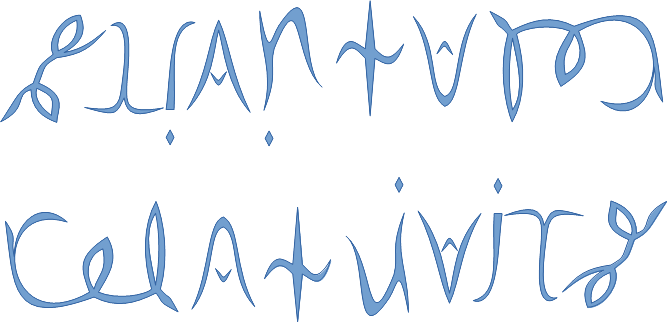See SRQM: QM from SR - The 4-Vector RoadMap (.html)
See SRQM: QM from SR - The 4-Vector RoadMap (.pdf)

SRQM 4-Vector : Four-Vector and Lorentz Scalar Diagram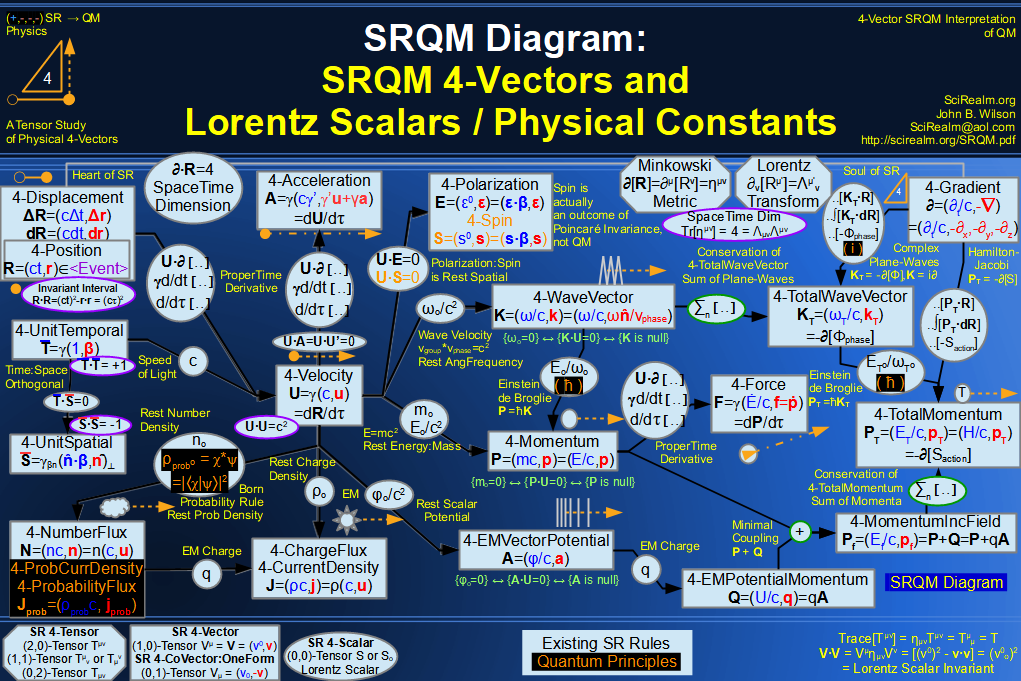SRQM + EM 4-Vector : Four-Vector and Lorentz Scalar Diagram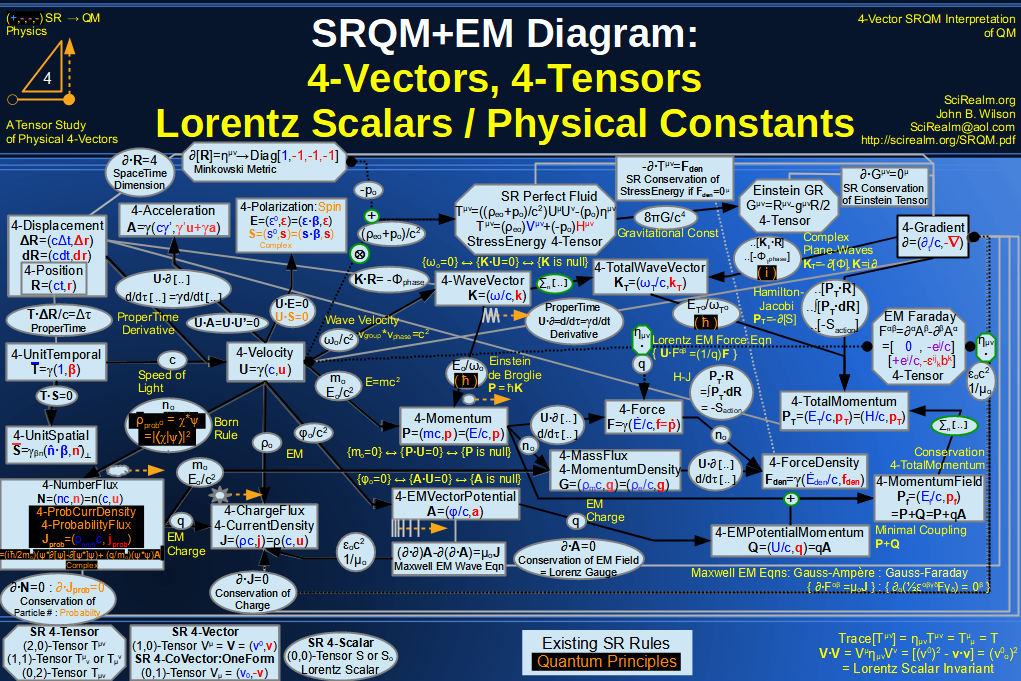SRQM + EM 4-Vector : Four-Vector and Lorentz Scalar Diagram With Tensor Invariants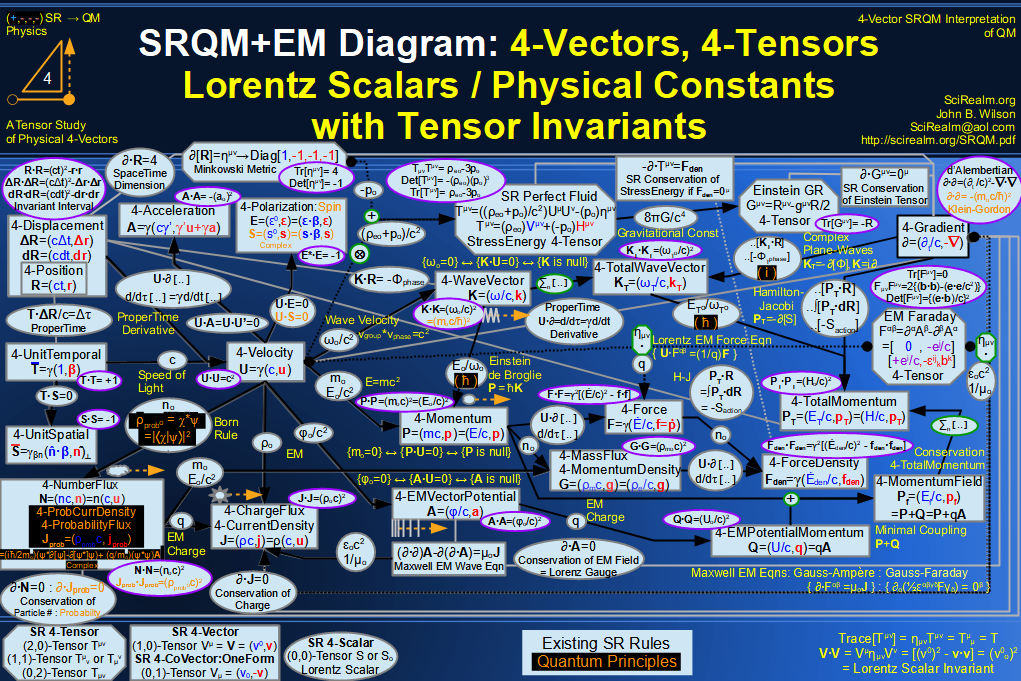SRQM 4-Vector : Four-Vector Stress-Energy & Projection Tensors Diagram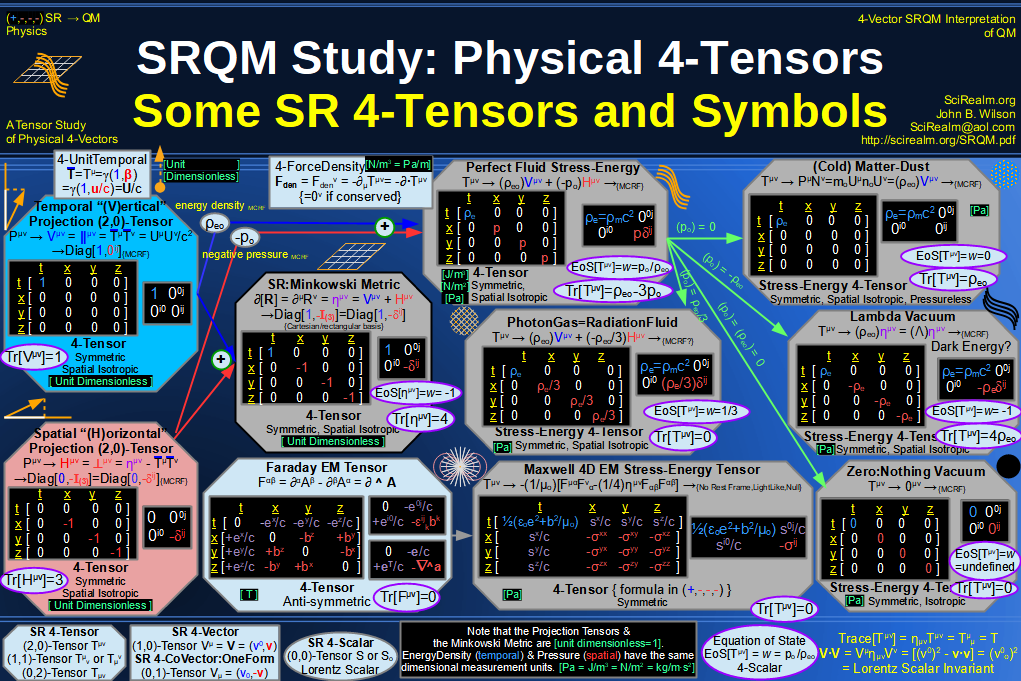SRQM 4-Vector : Four-Vector SR Quantum RoadMap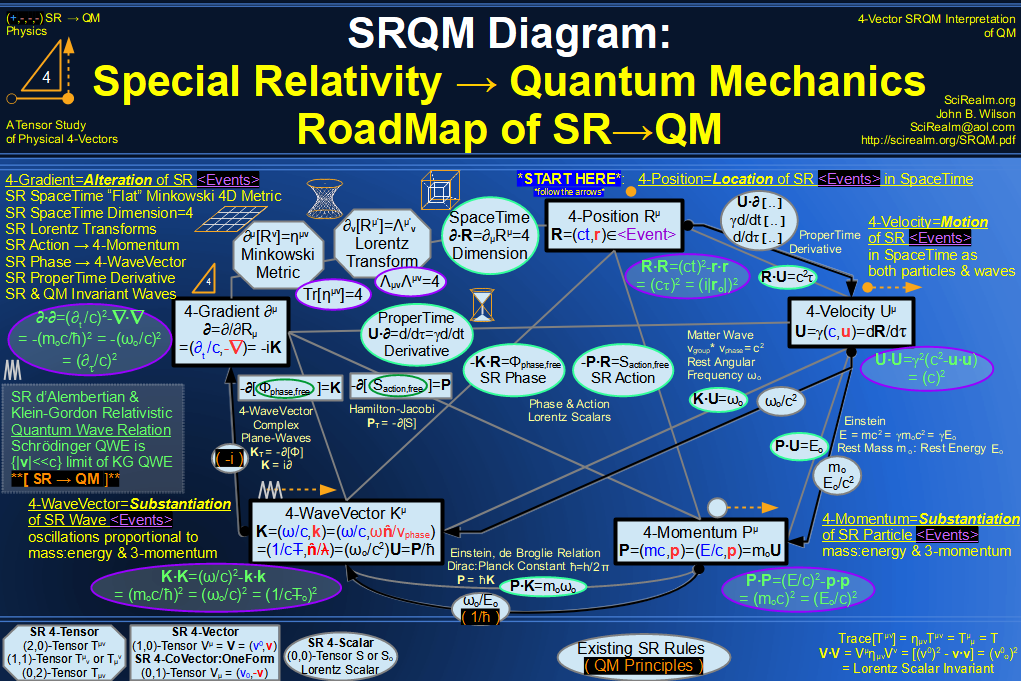SRQM + EM 4-Vector : Four-Vector SR Quantum RoadMap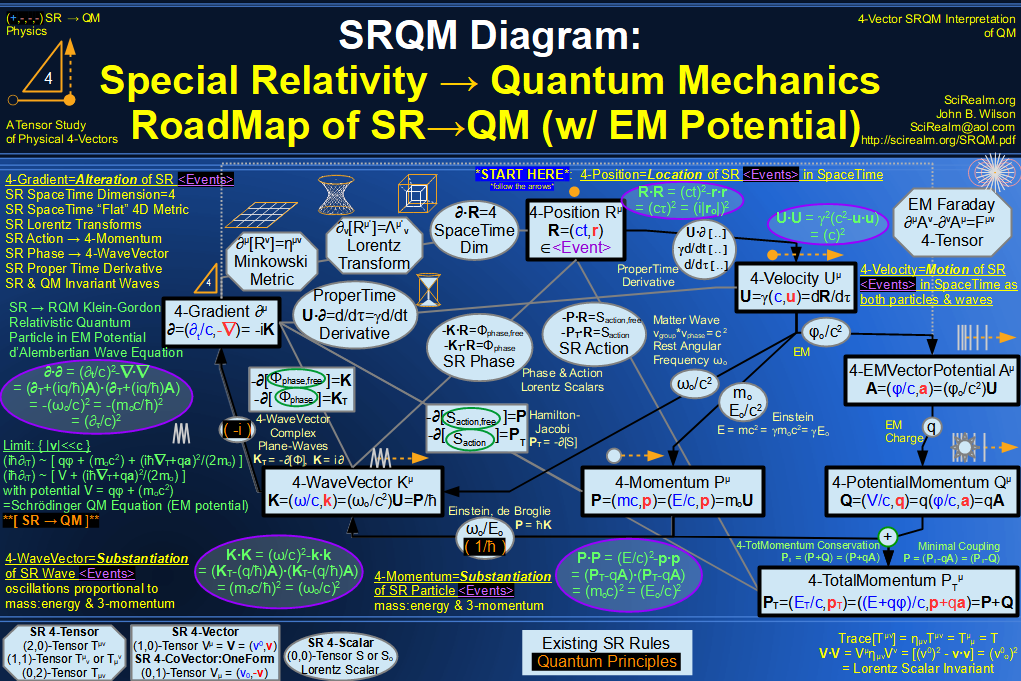SRQM + EM 4-Vector : Four-Vector SR Quantum RoadMap - Simple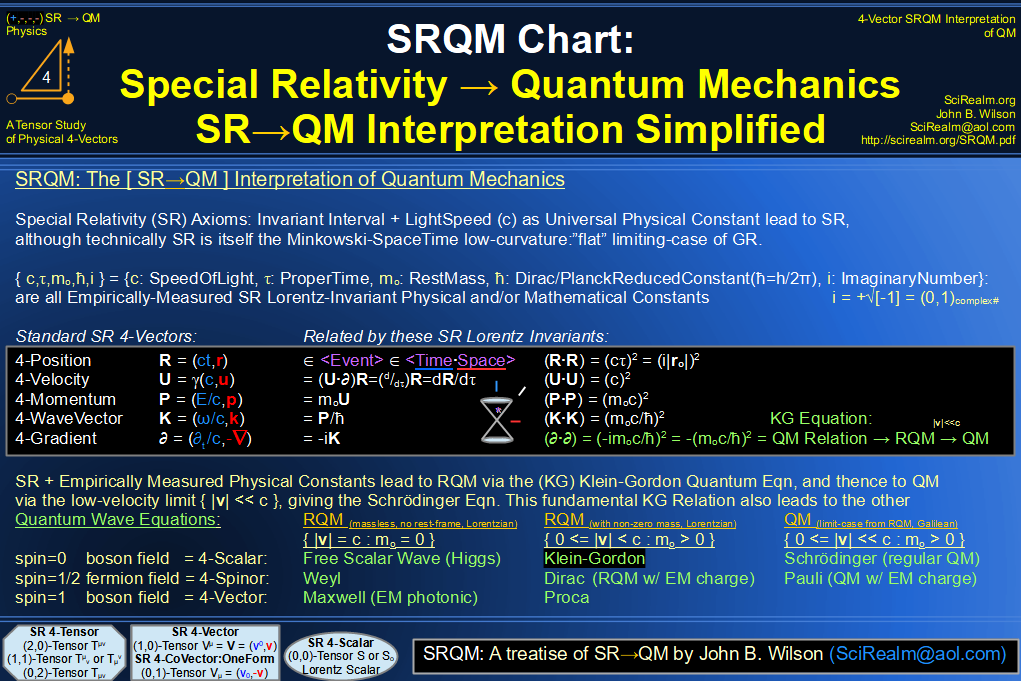SRQM 4-Vector : Four-Vector New Relativistic Quantum Paradigm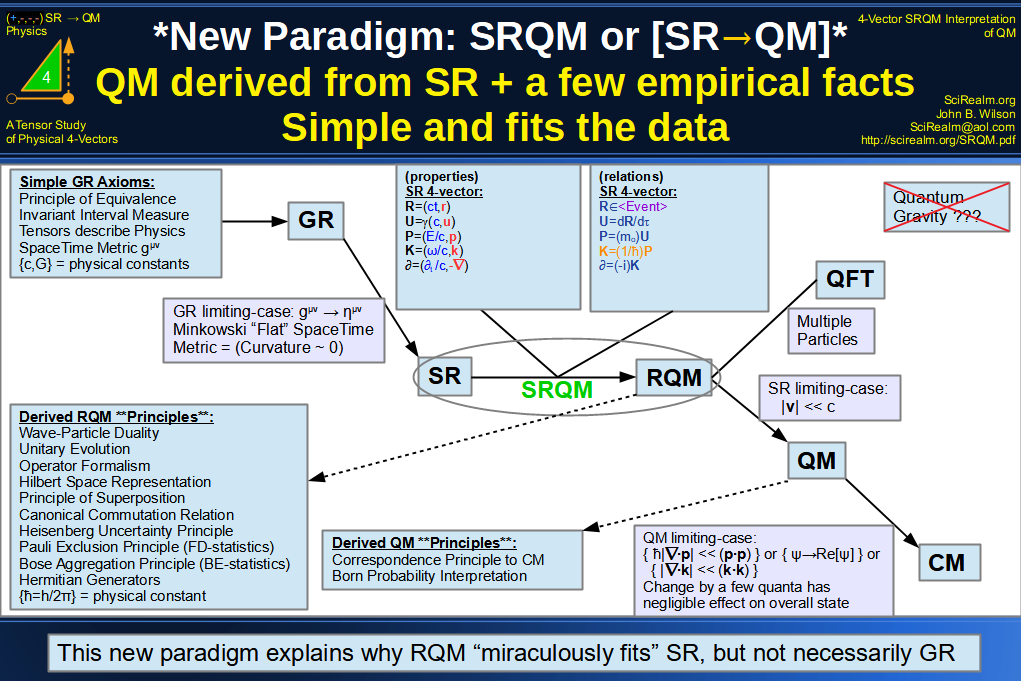SRQM + EM 4-Vector : Four-Vector New Relativistic Quantum Paradigm (with EM)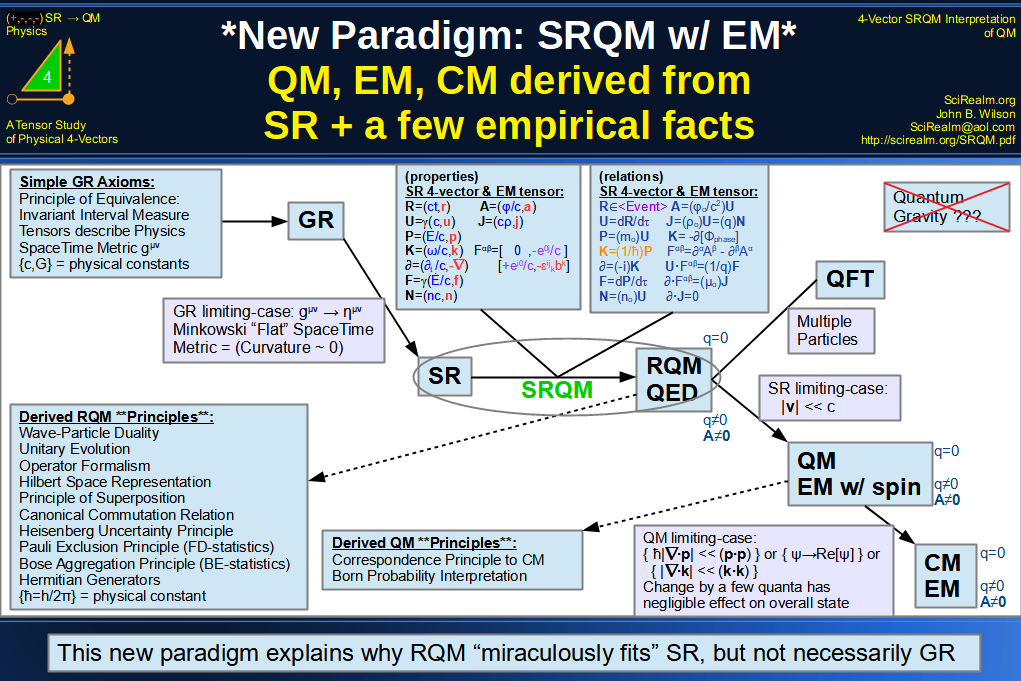SRQM 4-Vector : Four-Vector New Relativistic Quantum Paradigm - Venn Diagram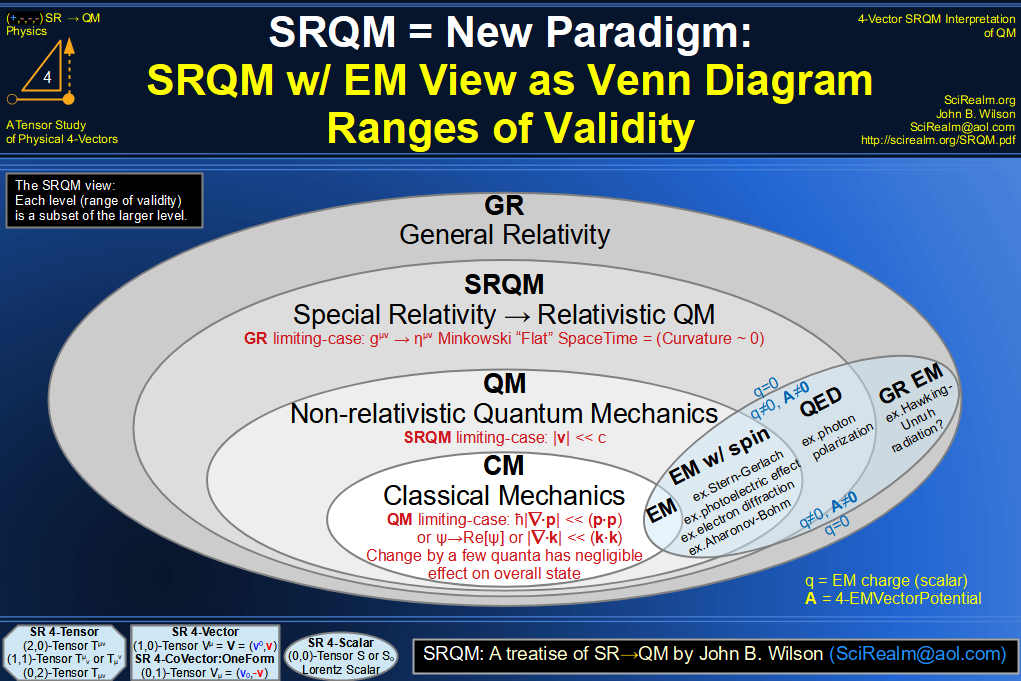SRQM 4-Vector : Four-Vector SpaceTime is 4D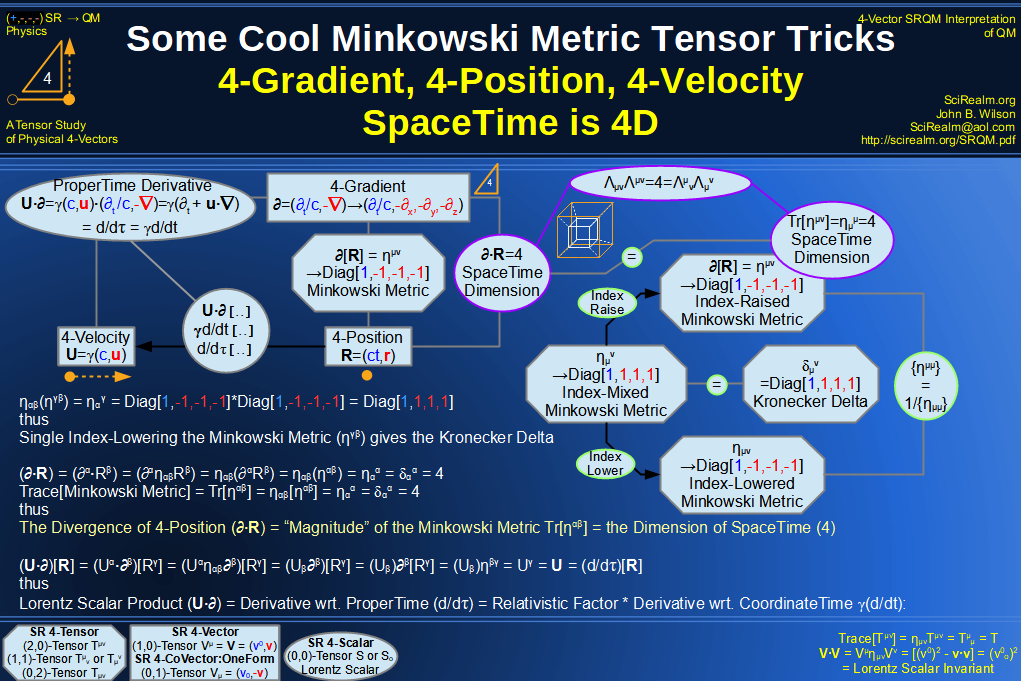SRQM 4-Vector : Four-Vector SpaceTime Orthogonality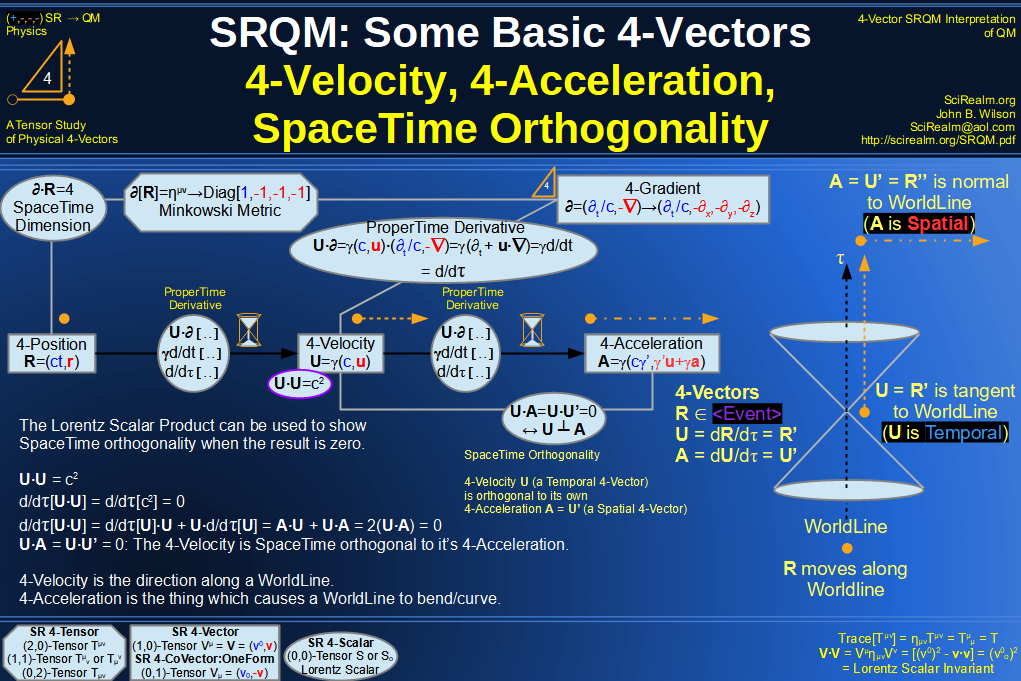SRQM 4-Vector : Four-Vector 4-Position, 4-Velocity, 4-Acceleration Diagram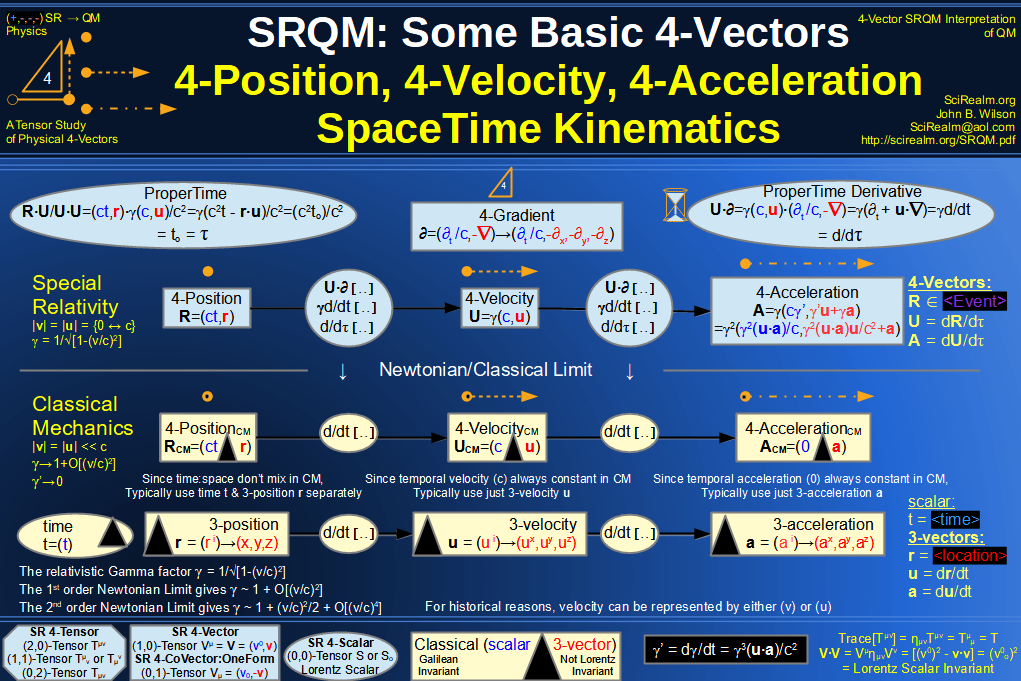SRQM 4-Vector : Four-Vector 4-Displacement, 4-Velocity, Relativity of Simultaneity Diagram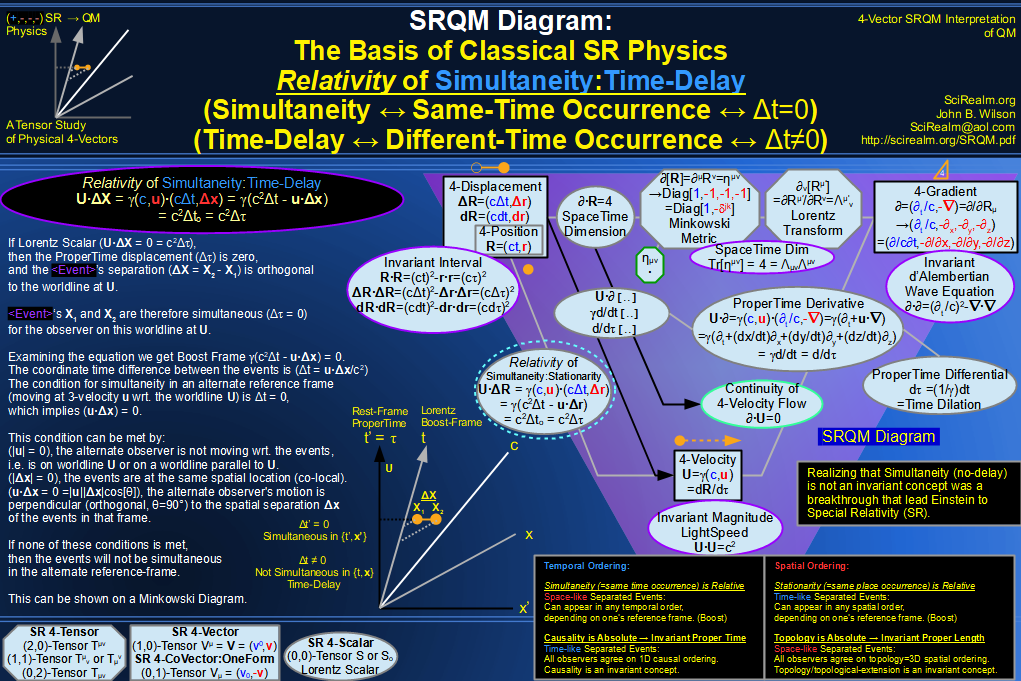SRQM 4-Vector : Four-Vector 4-Velocity, 4-Gradient, Time Dilation Diagram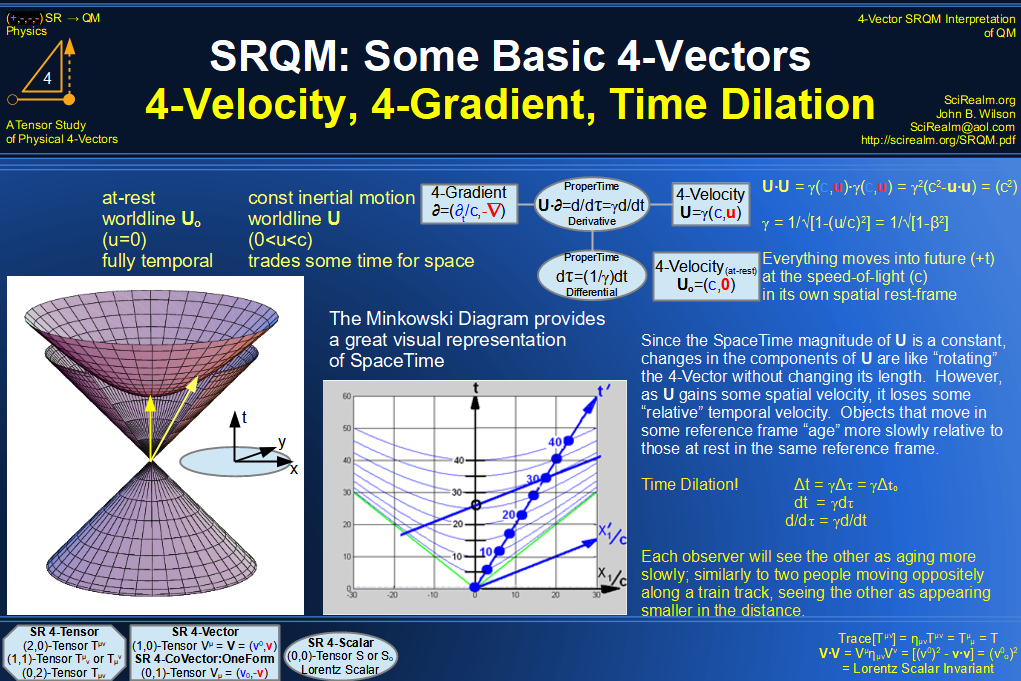SRQM 4-Vector : Four-Vector 4-Vector, 4-Velocity, 4-Momentum, E=mc^2 Diagram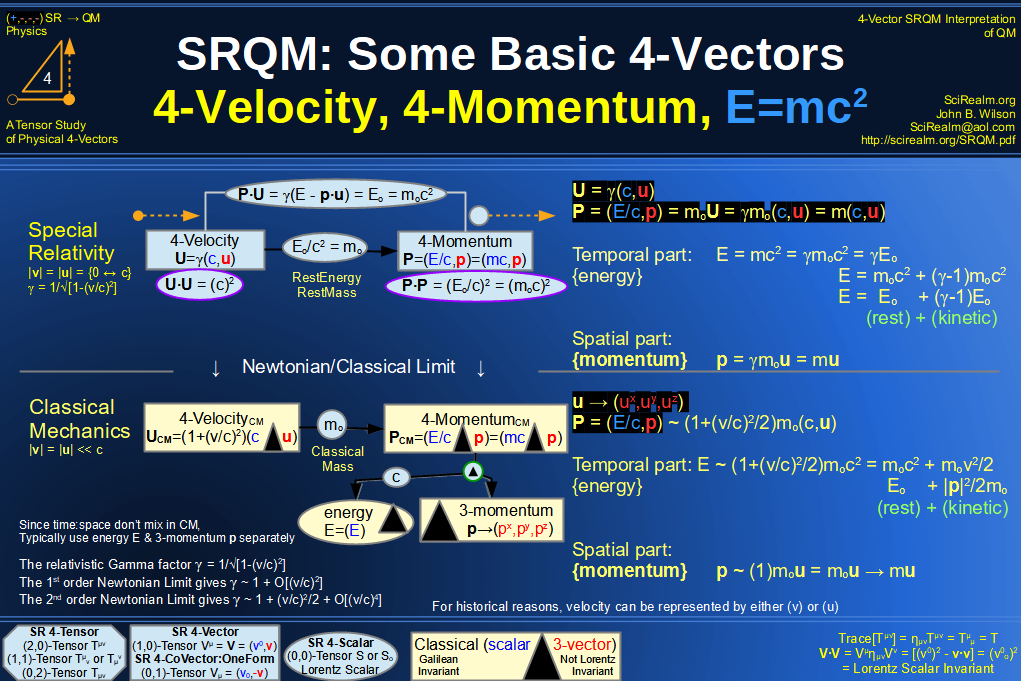SRQM 4-Vector : Four-Vector 4-Velocity, 4-WaveVector, Relativistic Doppler Effect Diagram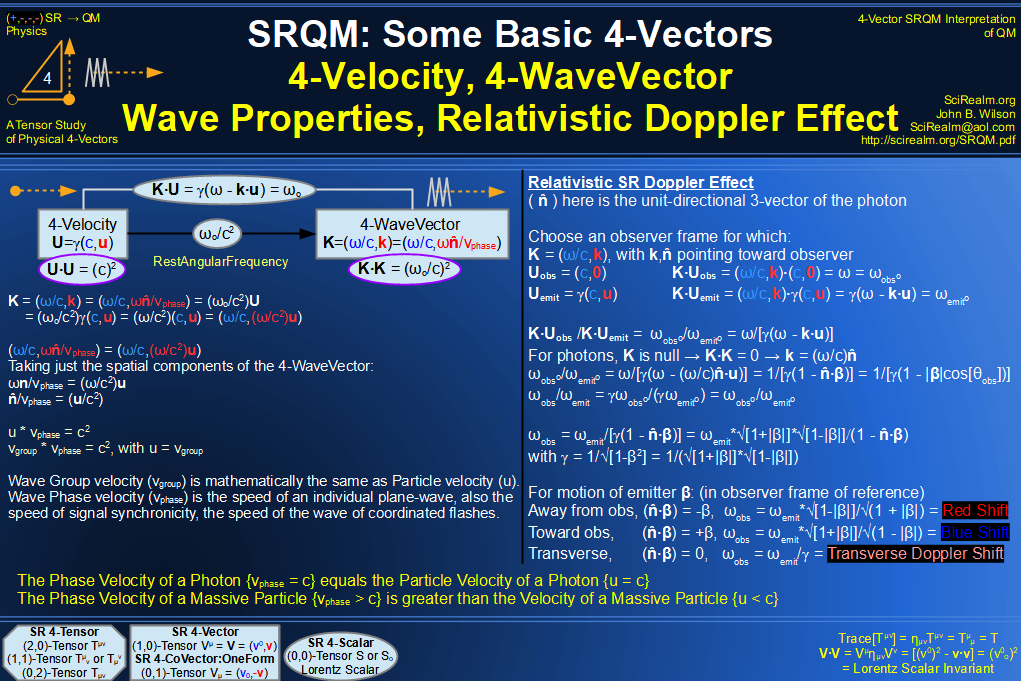SRQM 4-Vector : Four-Vector Wave-Particle Diagram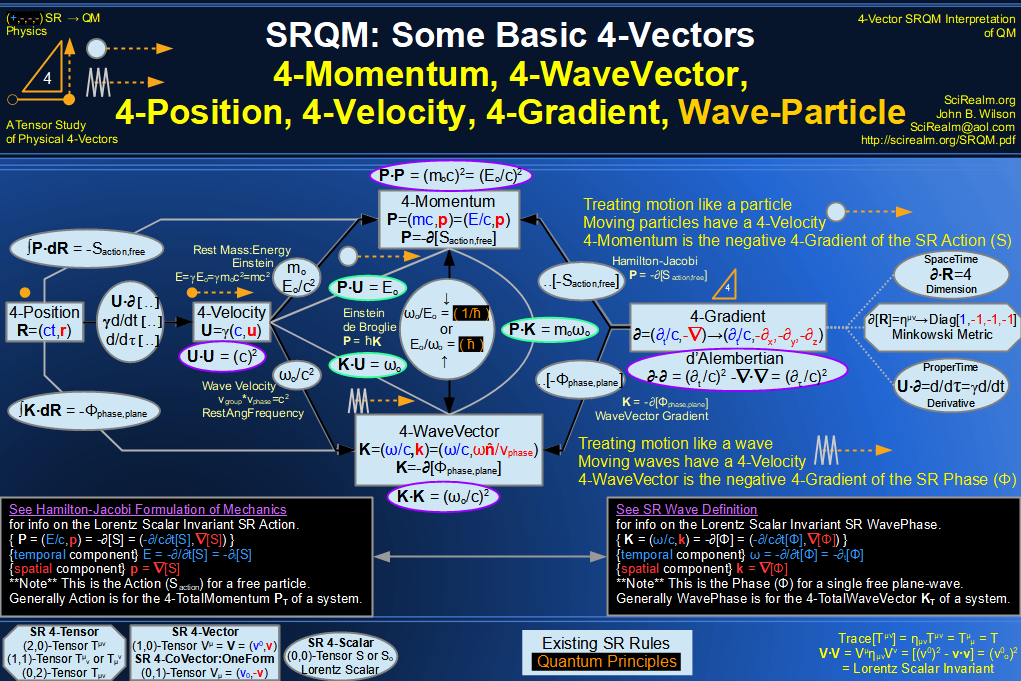SRQM 4-Vector : Four-Vector Compton Effect Diagram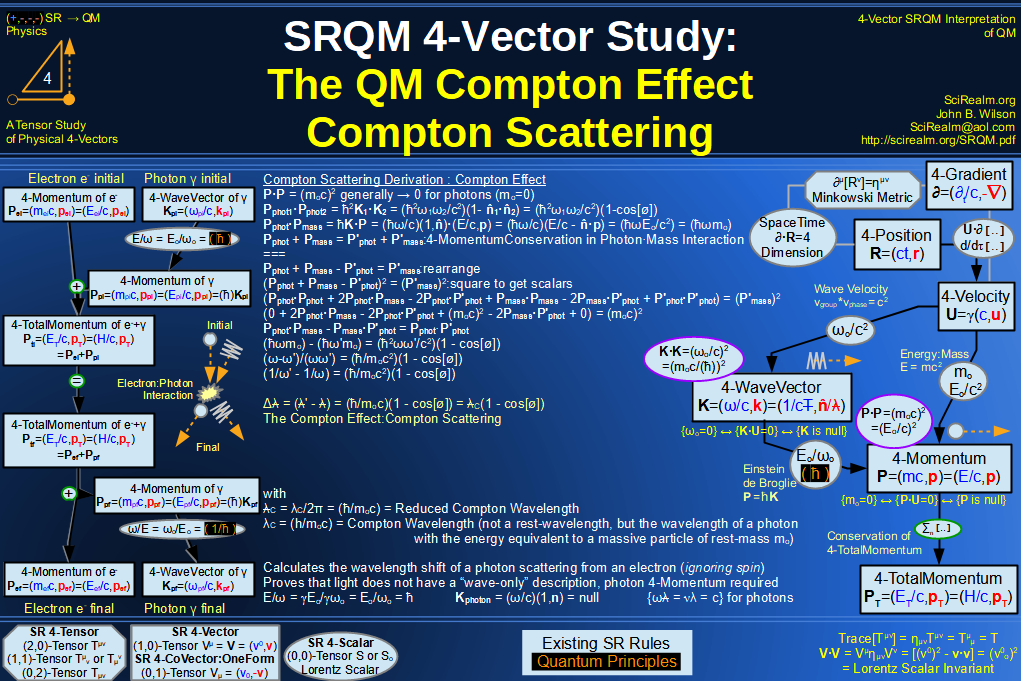SRQM 4-Vector : Four-Vector Aharonov-Bohm Effect Diagram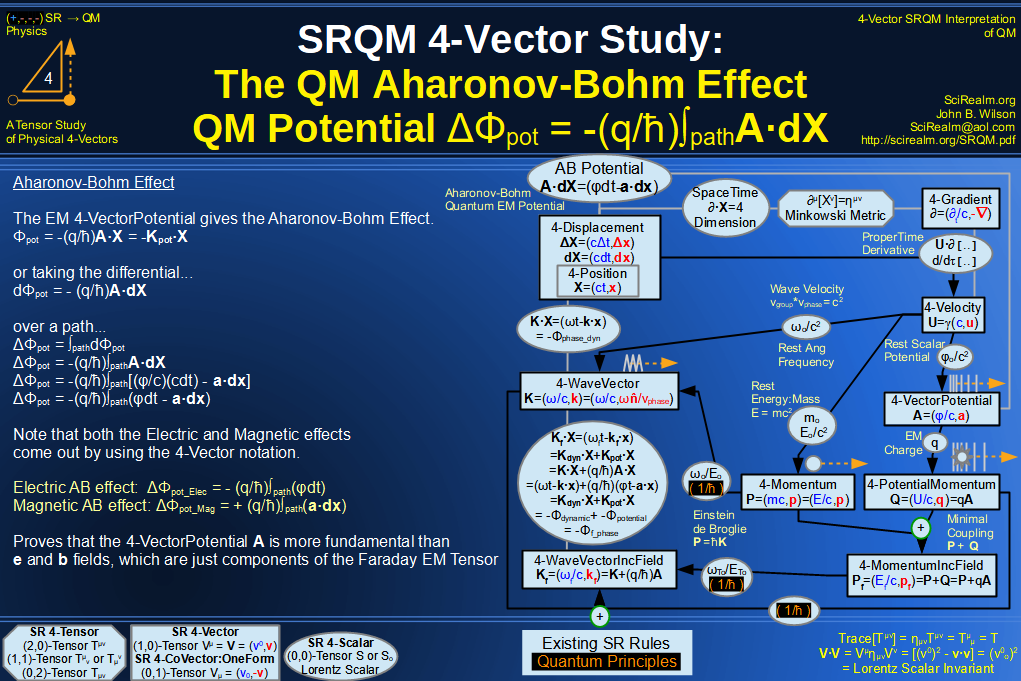SRQM 4-Vector : Four-Vector Josephson Junction Effect Diagram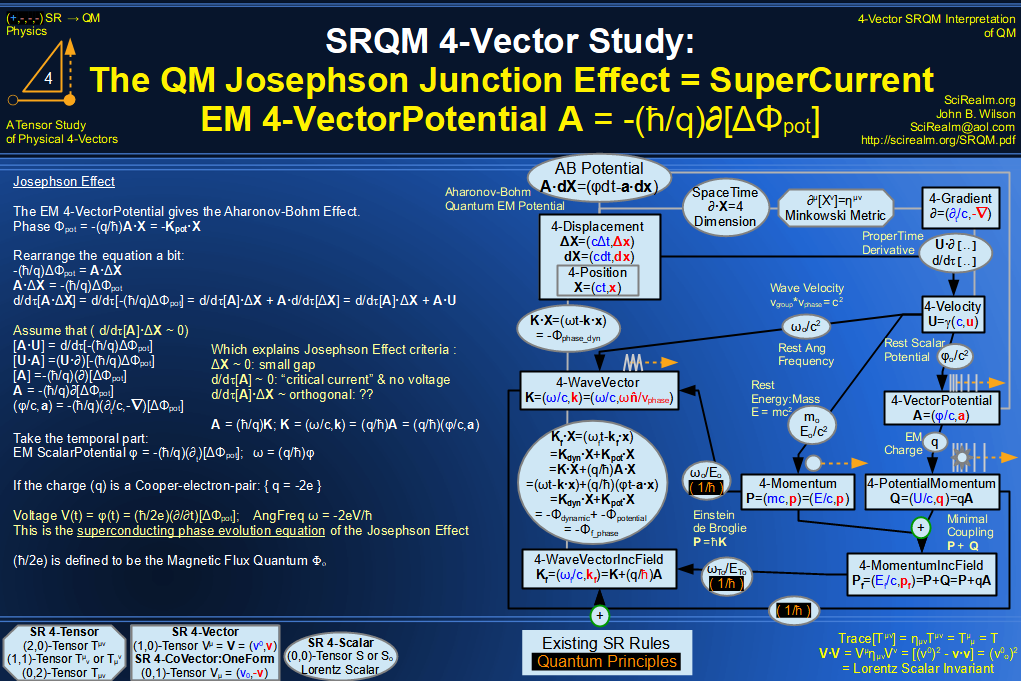SRQM 4-Vector : Four-Vector Hamilton-Jacobi vs Action, Josephson vs Aharonov-Bohm Diagram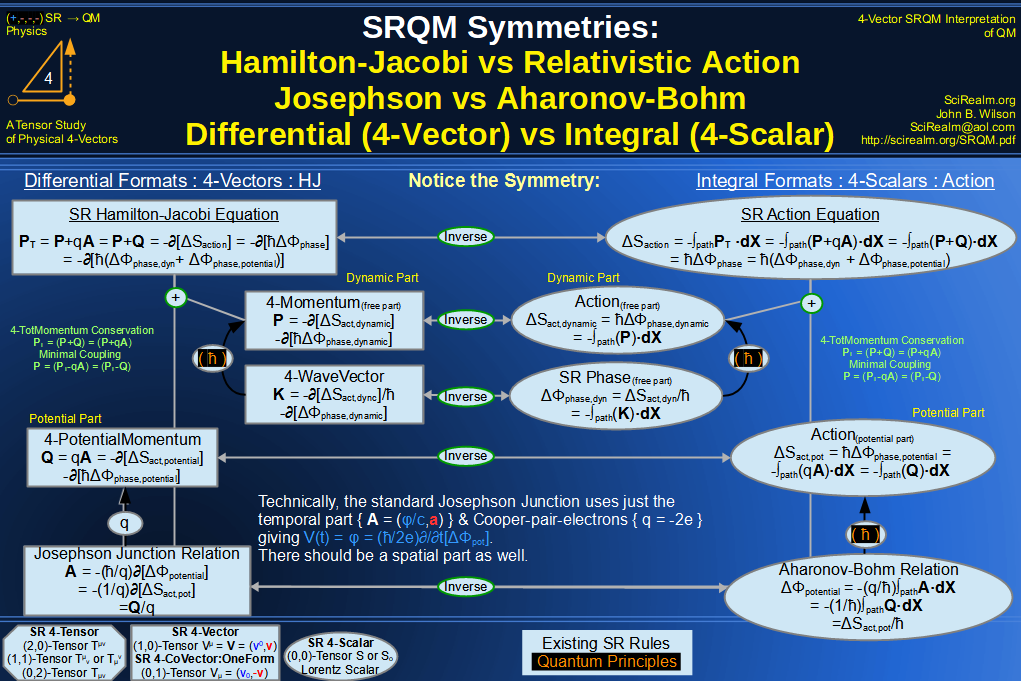SRQM 4-Vector : Four-Vector Motion of Lorentz Scalar Invariants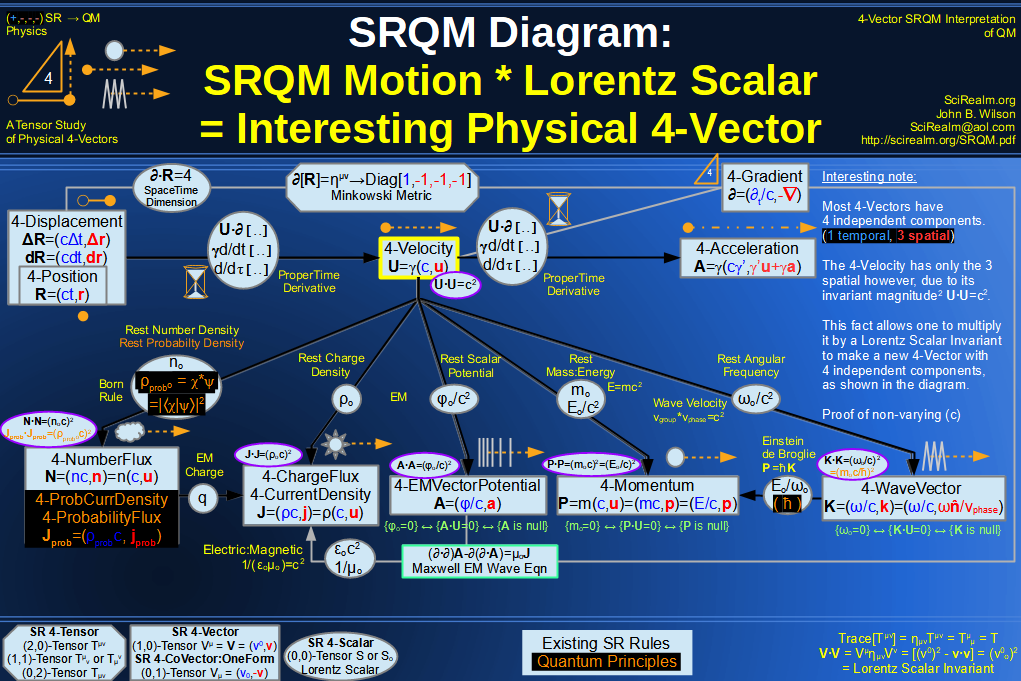SRQM 4-Vector : Four-Vector Motion of Lorentz Scalar Invariants, Conservation Laws & Continuity Equations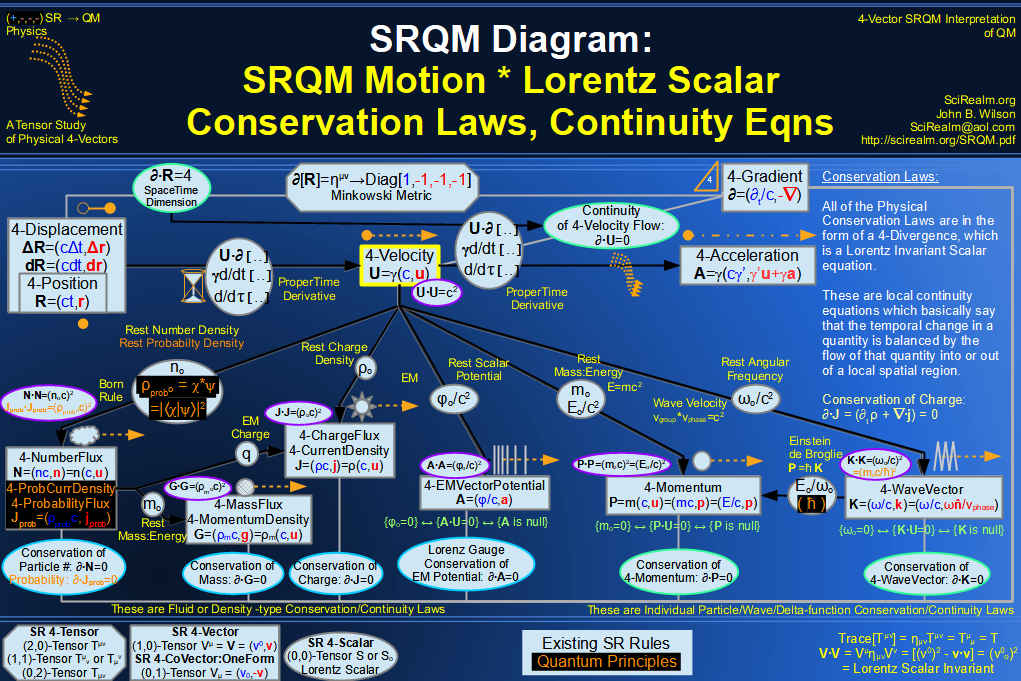SRQM 4-Vector : Four-Vector Speed of Light (c)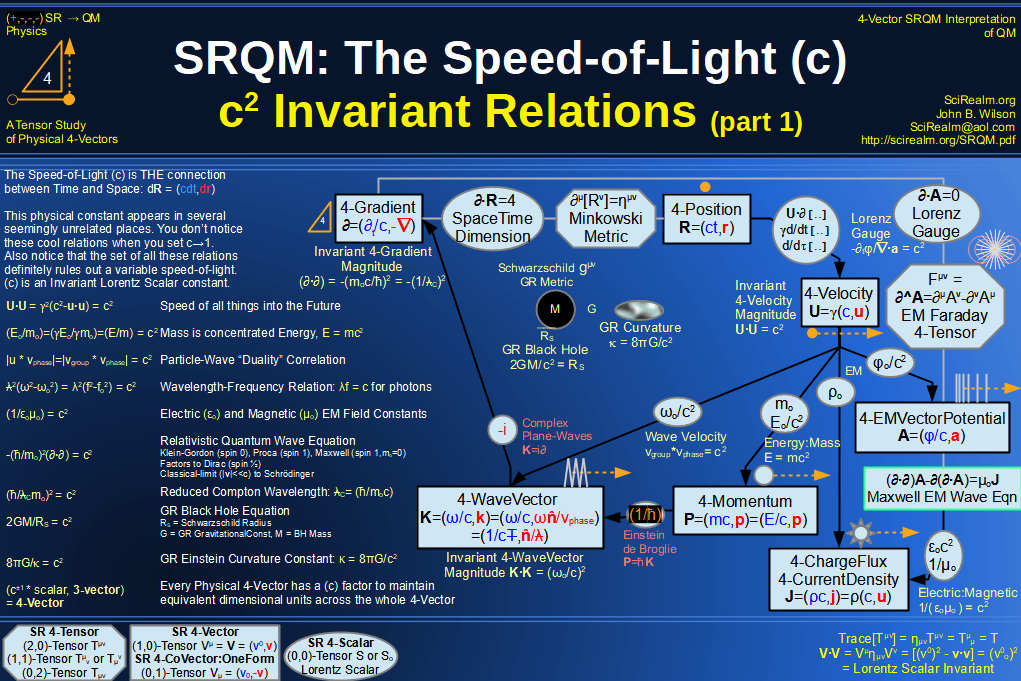SRQM 4-Vector : Four-Vector Minimal Coupling Conservation of 4-TotalMomentum)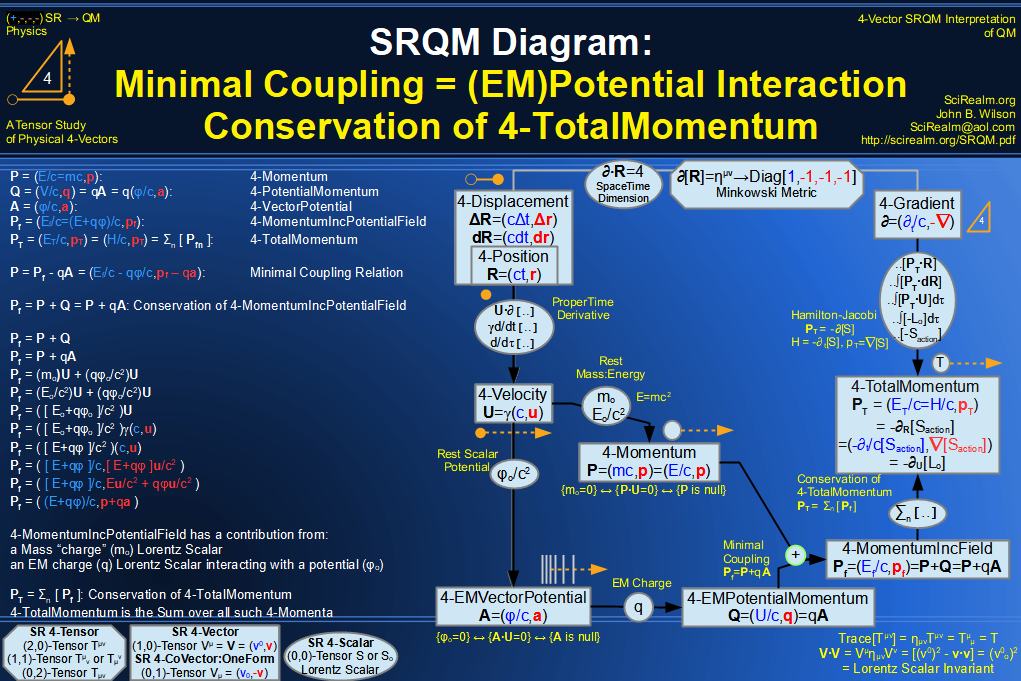SRQM 4-Vector : Four-Vector Relativistic Action (S) Diagram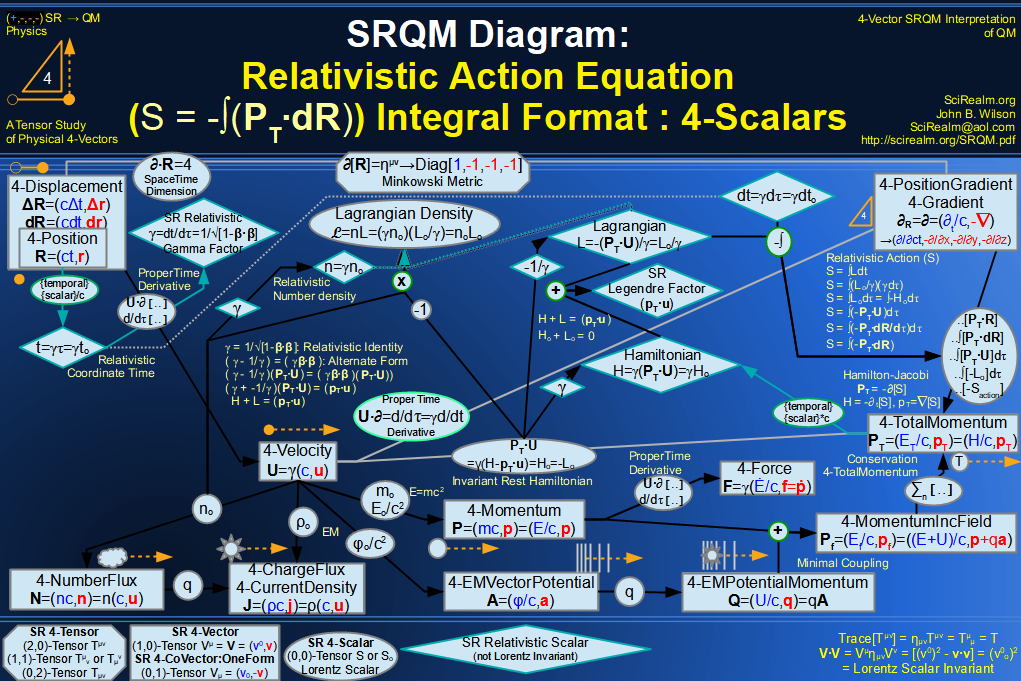SRQM 4-Vector : Four-Vector Relativistic Lagrangian Hamiltonian Diagram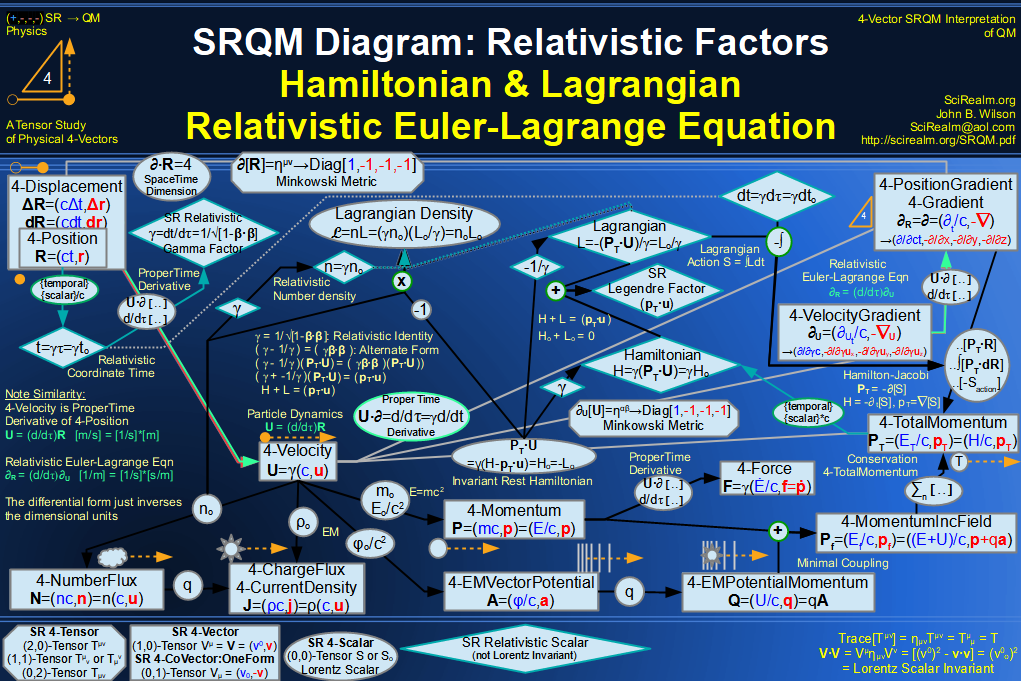SRQM 4-Vector : Four-Vector Relativistic Euler-Lagrange Equation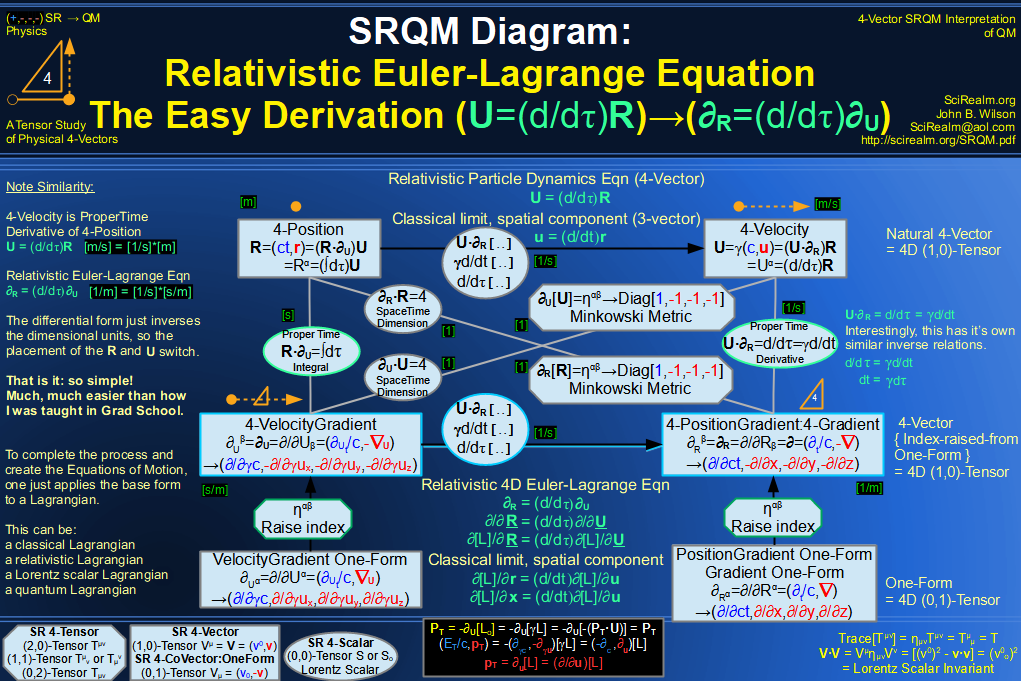SRQM 4-Vector : Four-Vector Relativistic EM Equations of Motion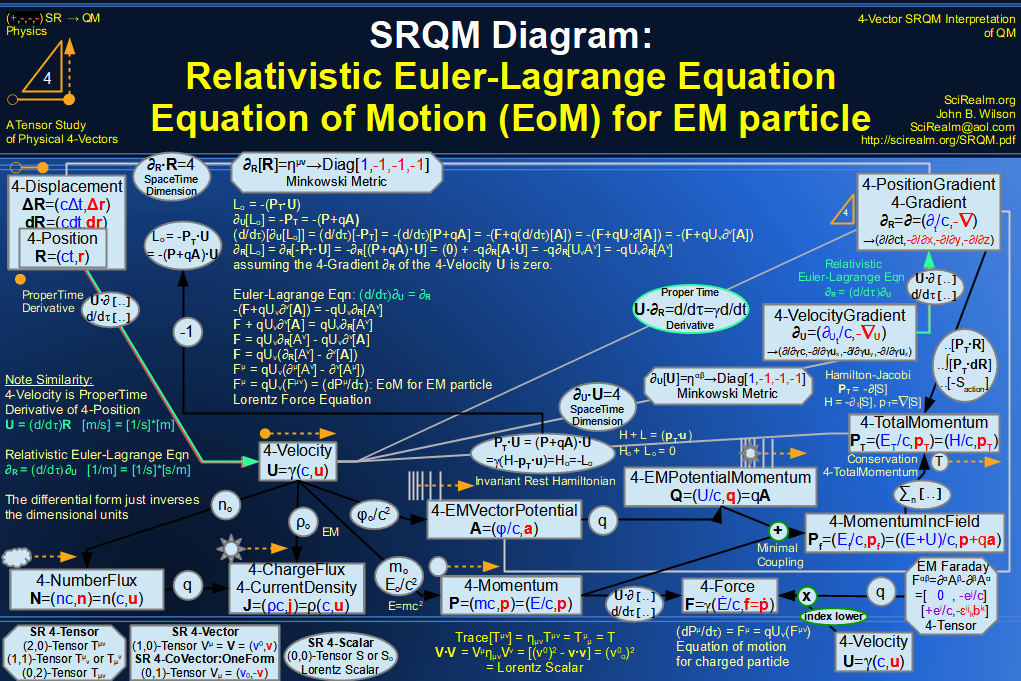SRQM 4-Vector : Four-Vector Einstein-de Broglie Relation hbar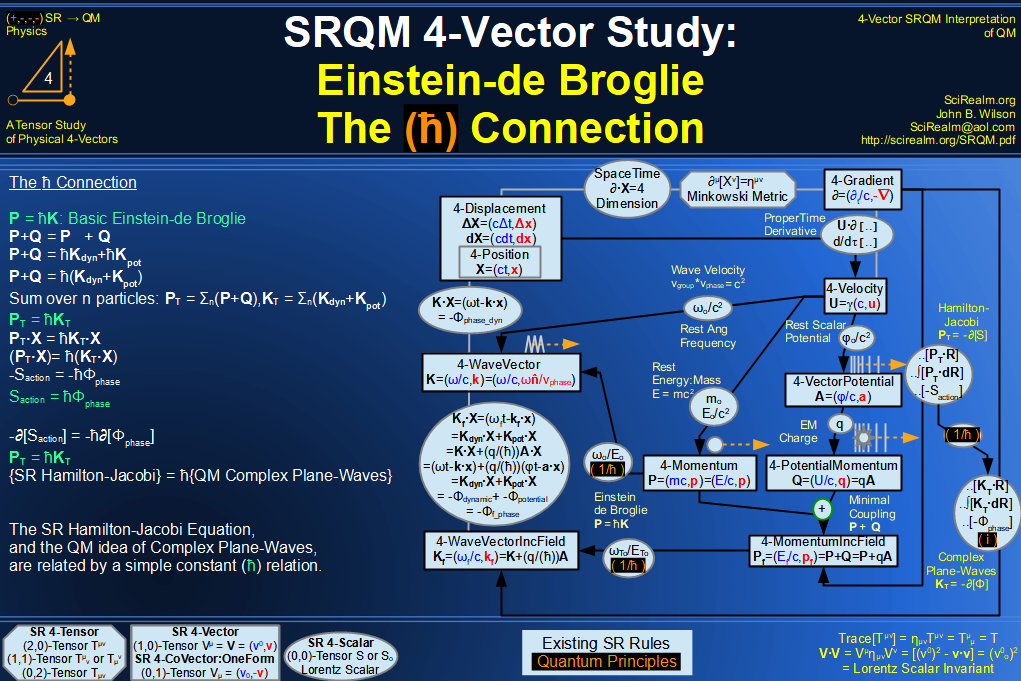SRQM 4-Vector : Four-Vector Quantum Canonical Commutation Relation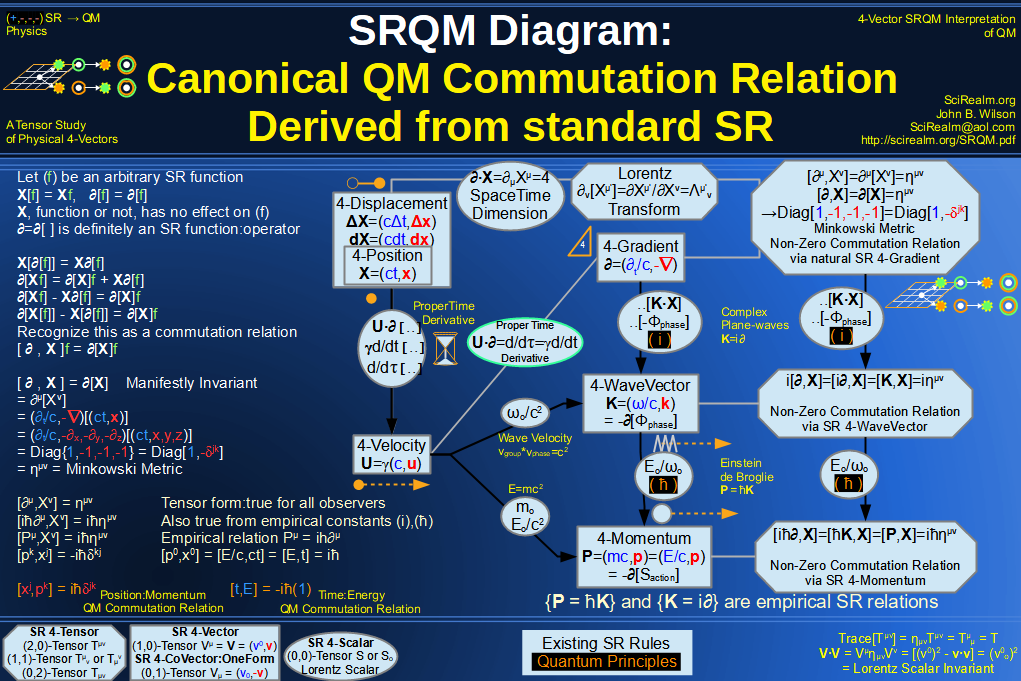SRQM 4-Vector : Four-Vector QM Schroedinger Relation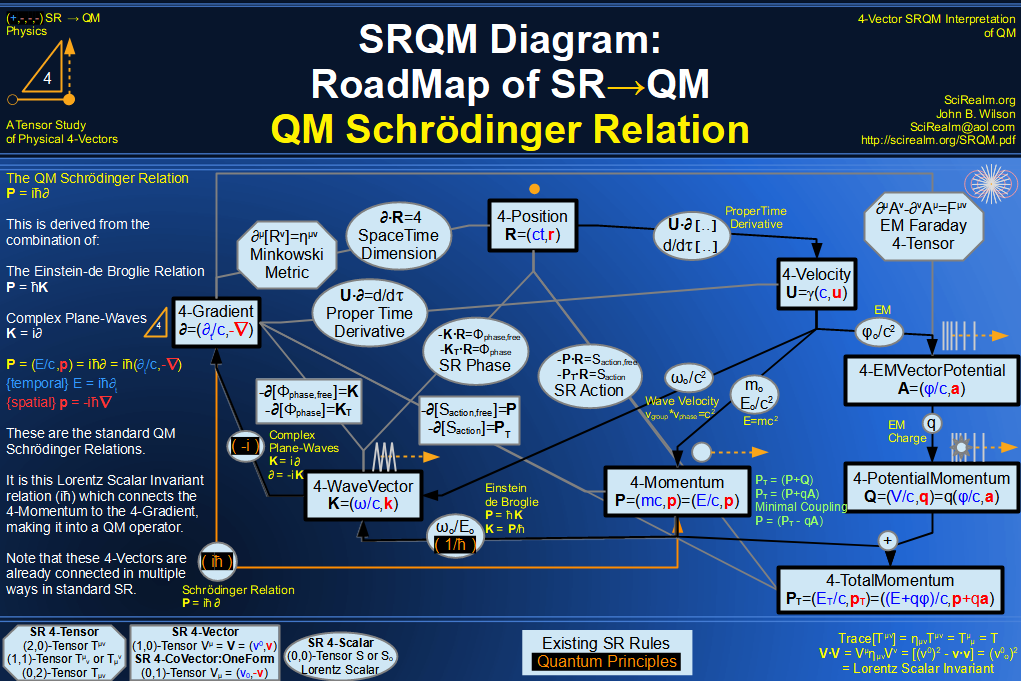SRQM 4-Vector : Four-Vector Quantum Probability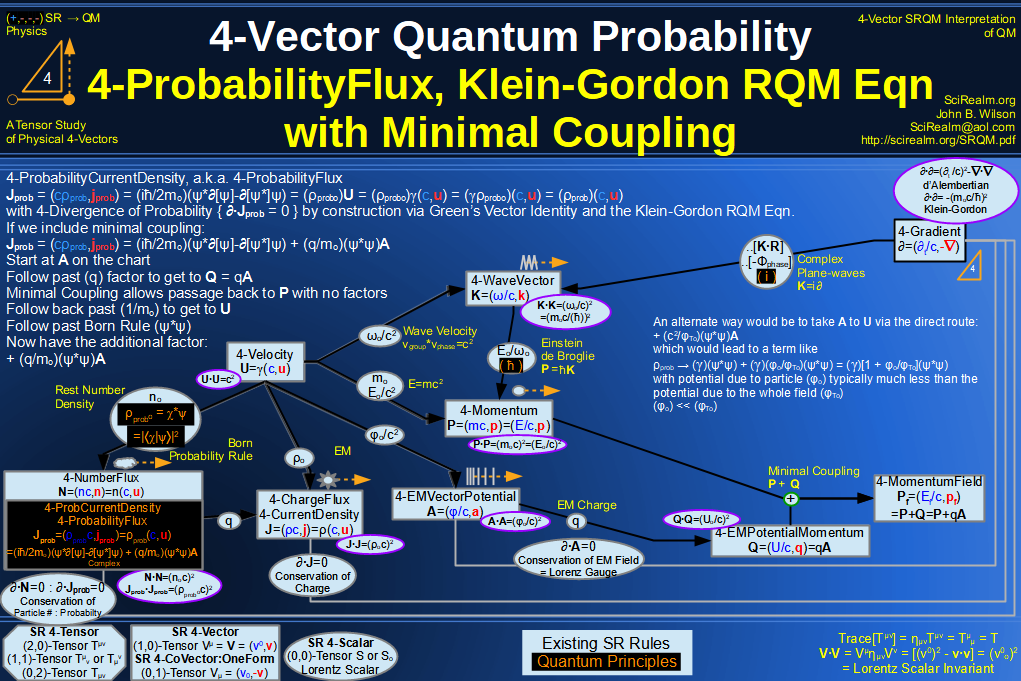SRQM 4-Vector : Four-Vector CPT Theorem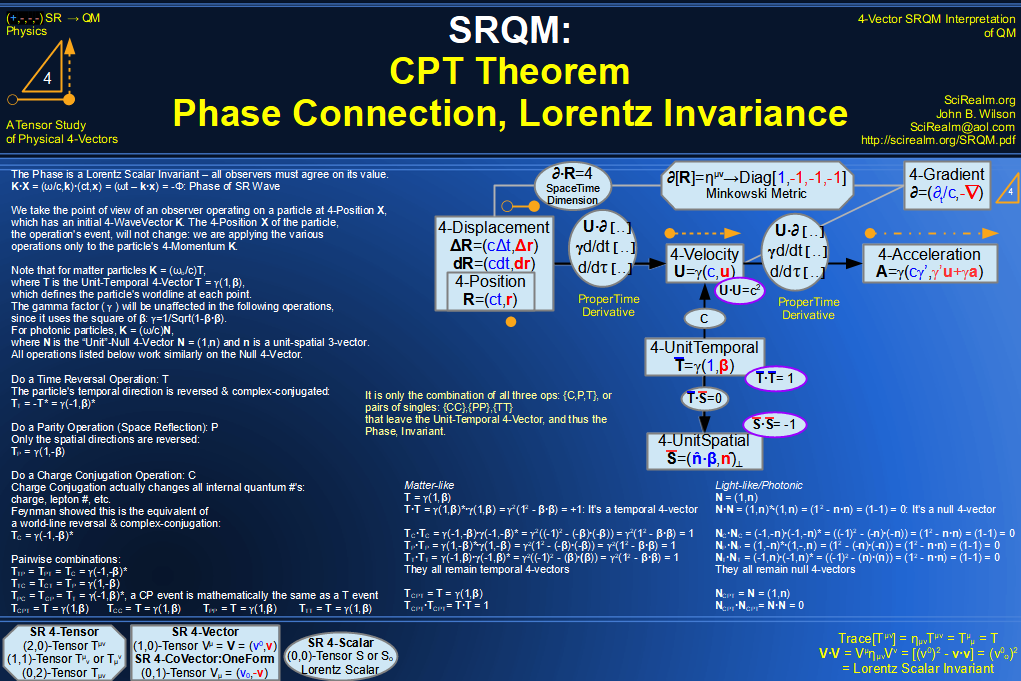SRQM 4-Vector : Four-Vector Lorentz Transforms Connection Map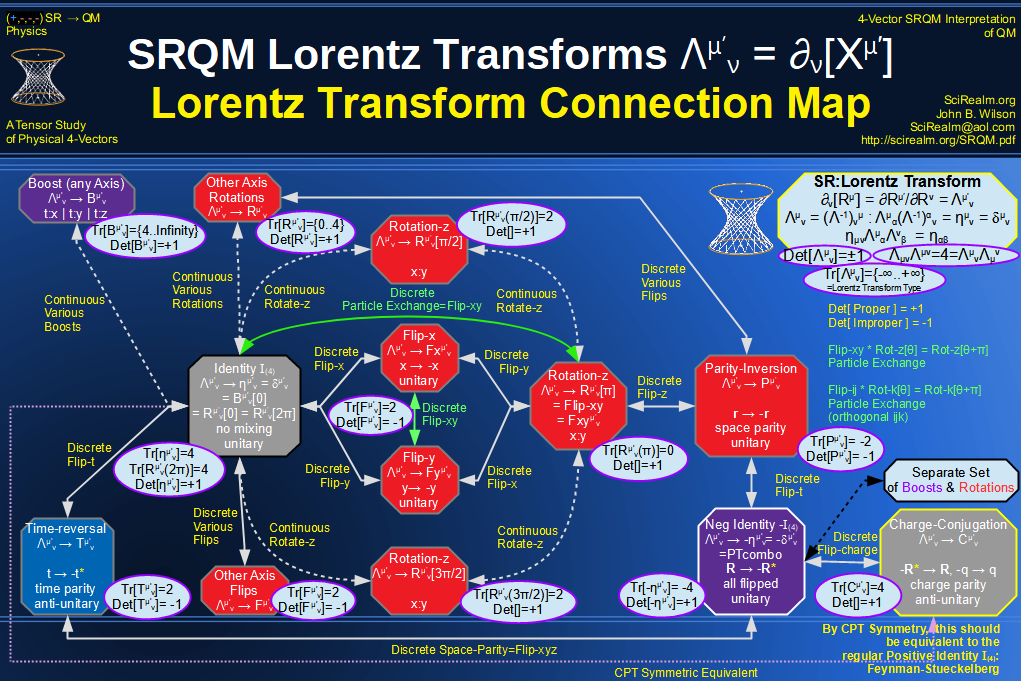SRQM 4-Vector : Four-Vector Lorentz Discrete Transforms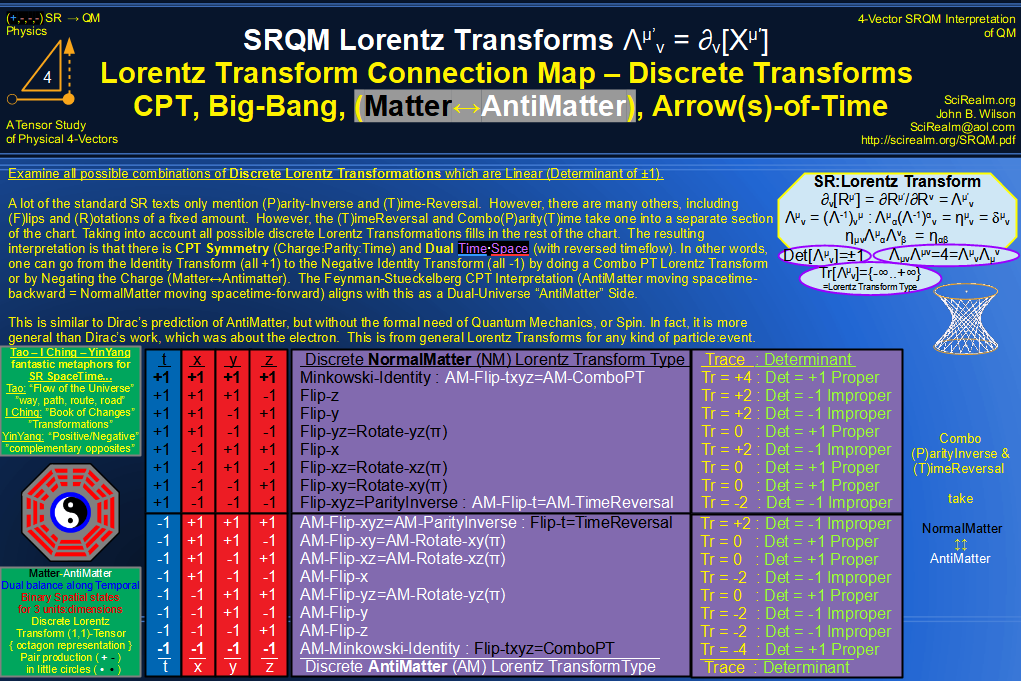SRQM 4-Vector : Four-Vector Lorentz Transforms - Trace Identification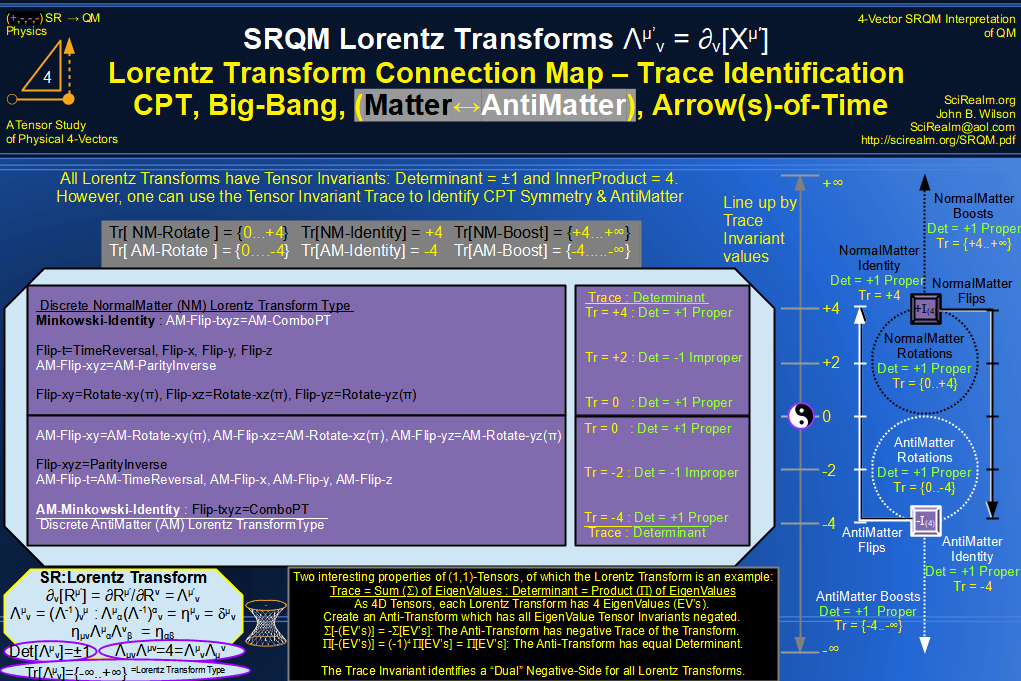SRQM 4-Vector : Four-Vector Lorentz Lorentz Transforms-Interpretations
CPT Symmetry, Baryon Asymmetry Problem Solution, Matter-Antimatter Symmetry Solution, Arrow-of-Time Problem Solution, Big-Bang!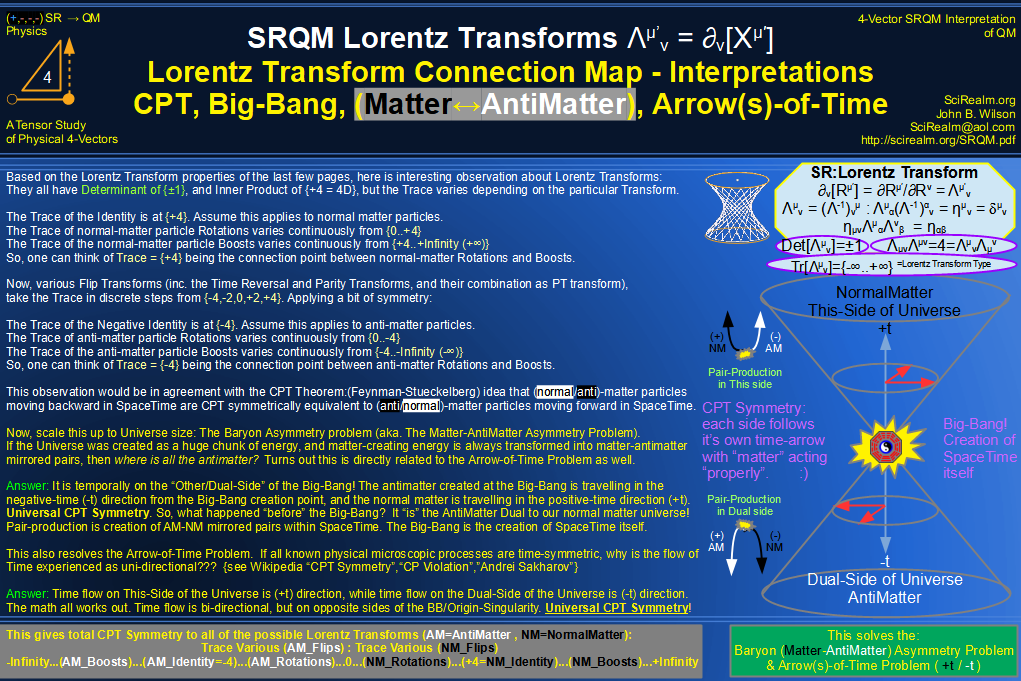See SRQM: QM from SR - The 4-Vector RoadMap (.html)
See SRQM: QM from SR - The 4-Vector RoadMap (.pdf)The Science Realm: John's Virtual Sci-Tech Universe John's Science & Math Stuff: | About John | Send email to John 4-Vectors | Ambigrams | Antipodes | Covert Ops Fragments | Cyrillic Projector | Forced Induction (Sums Of Powers Of Integers) | Fractals | Frontiers | JavaScript Graphics | Kid Science | Kryptos | Philosophy | Photography | Prime Sieve | QM from SR | QM from SR-Simple RoadMap | SR 4-Vector & Tensor Calculator | Quantum Phase | Quotes | RuneQuest Cipher Challenge | Scientific Calculator (complex capable) | Secret Codes & Ciphers | Science+Math | Sci-Pan Pantheist Poems | Stereograms | SuperMagicSqr4x4 | Turkish Grammar | Quantum Mechanics is derivable from Special Relativity See SRQM - QM from SR - Simple RoadMap (.html) See SRQM - QM from SR - Simple RoadMap (.pdf)